# RS Aggarwal Solutions for Class 9 Maths Chapter 18: Mean, Median and Mode of Ungrouped Data

The highly experienced faculties at BYJU’S have devised detailed exercise-wise solutions for the students to improve their conceptual knowledge. The RS Aggarwal Solutions contain steps in a descriptive manner that comes under Chapter 18 Mean, Median and Mode of Ungrouped Data of Class 9 textbook. Our subject experts have followed the latest syllabus while creating the solutions and is framed according to the exam pattern of the CBSE board.

By solving the questions of Chapter 18 by using RS Aggarwal Solutions, the students will be able to understand concepts and their weightage in the exam. The PDF of solutions is made available so that the students can download and use them as a resource to score well. RS Aggarwal Solutions for Class 9 Chapter 18 Mean, Median and Mode of Ungrouped Data are provided here.

## RS Aggarwal Solutions for Class 9 Maths Chapter 18: Mean, Median and Mode of Ungrouped Data Download PDF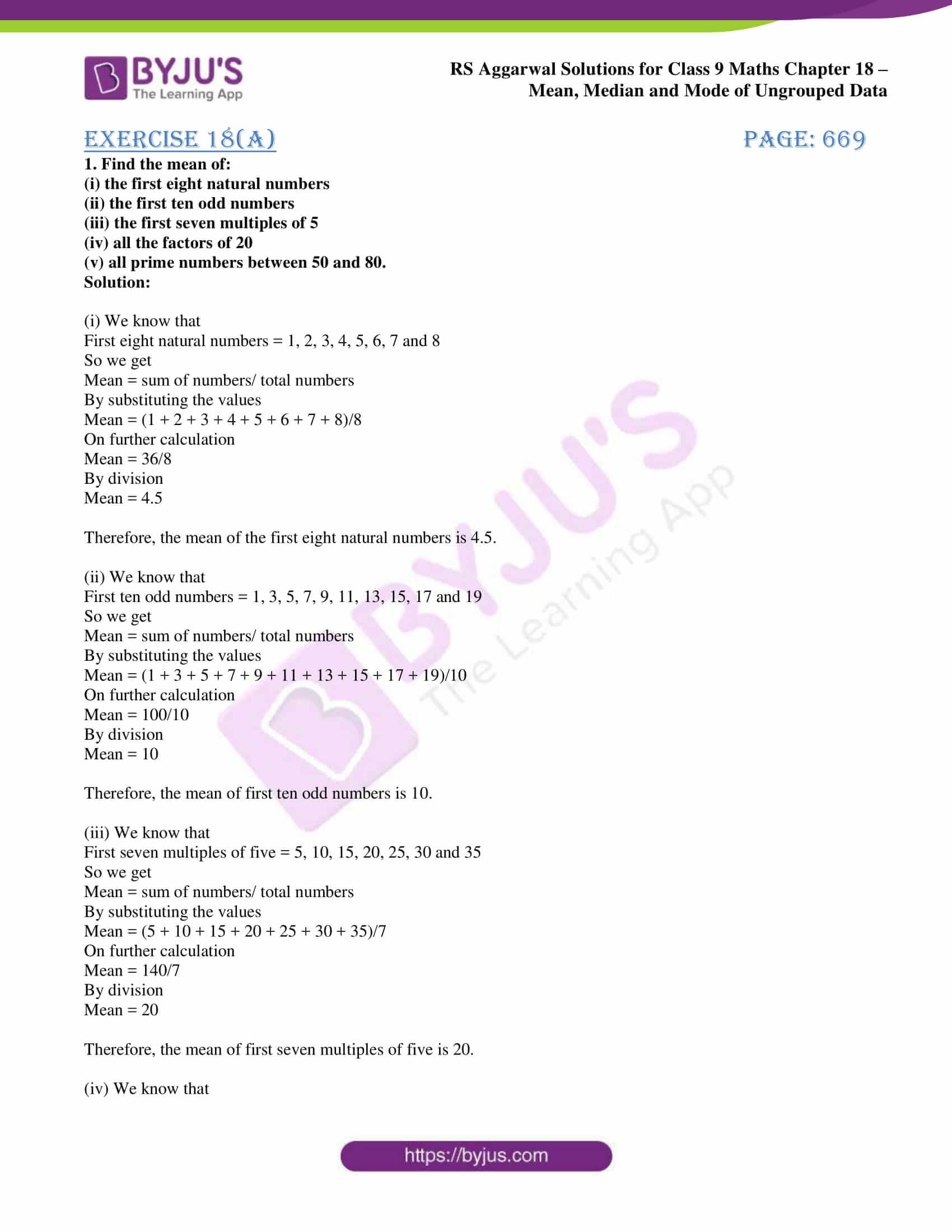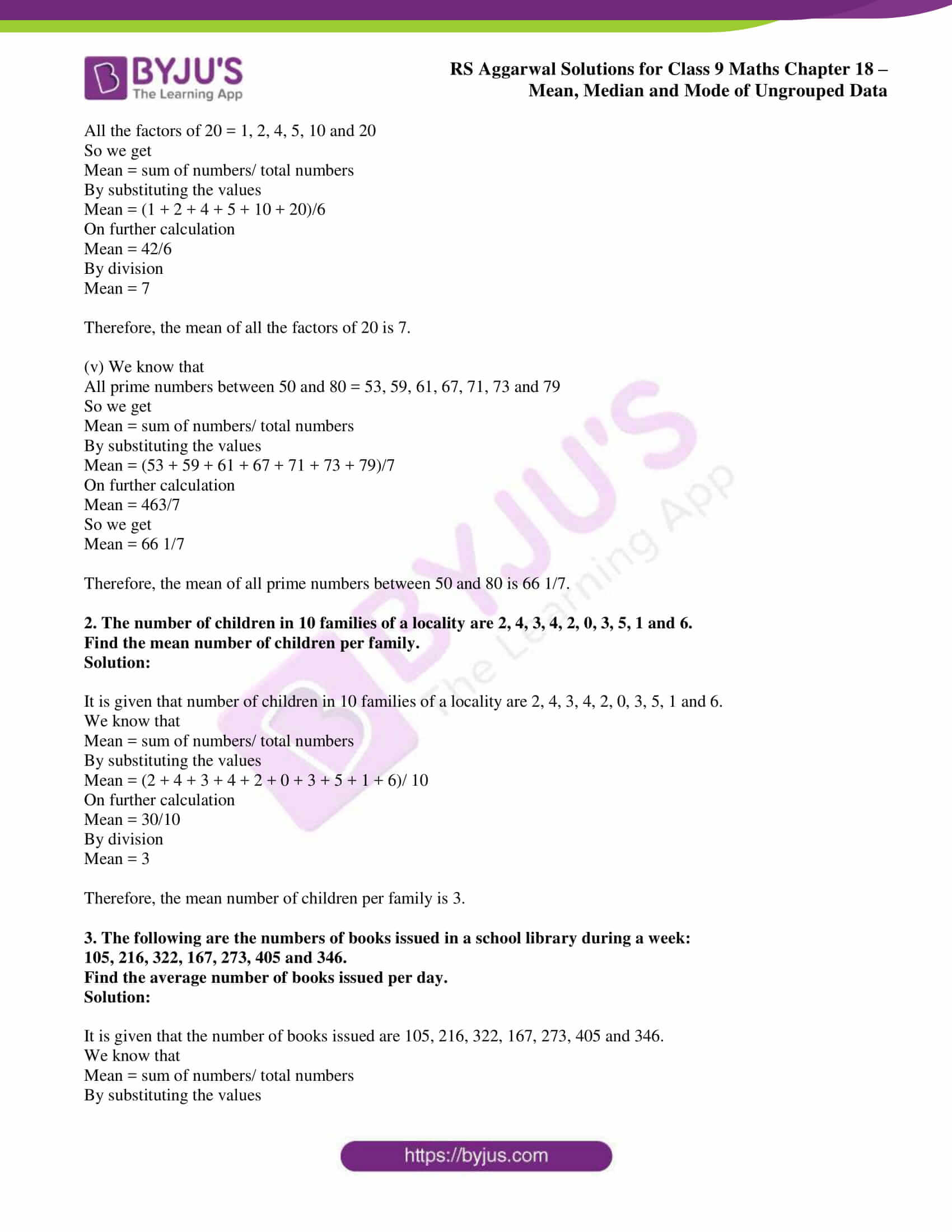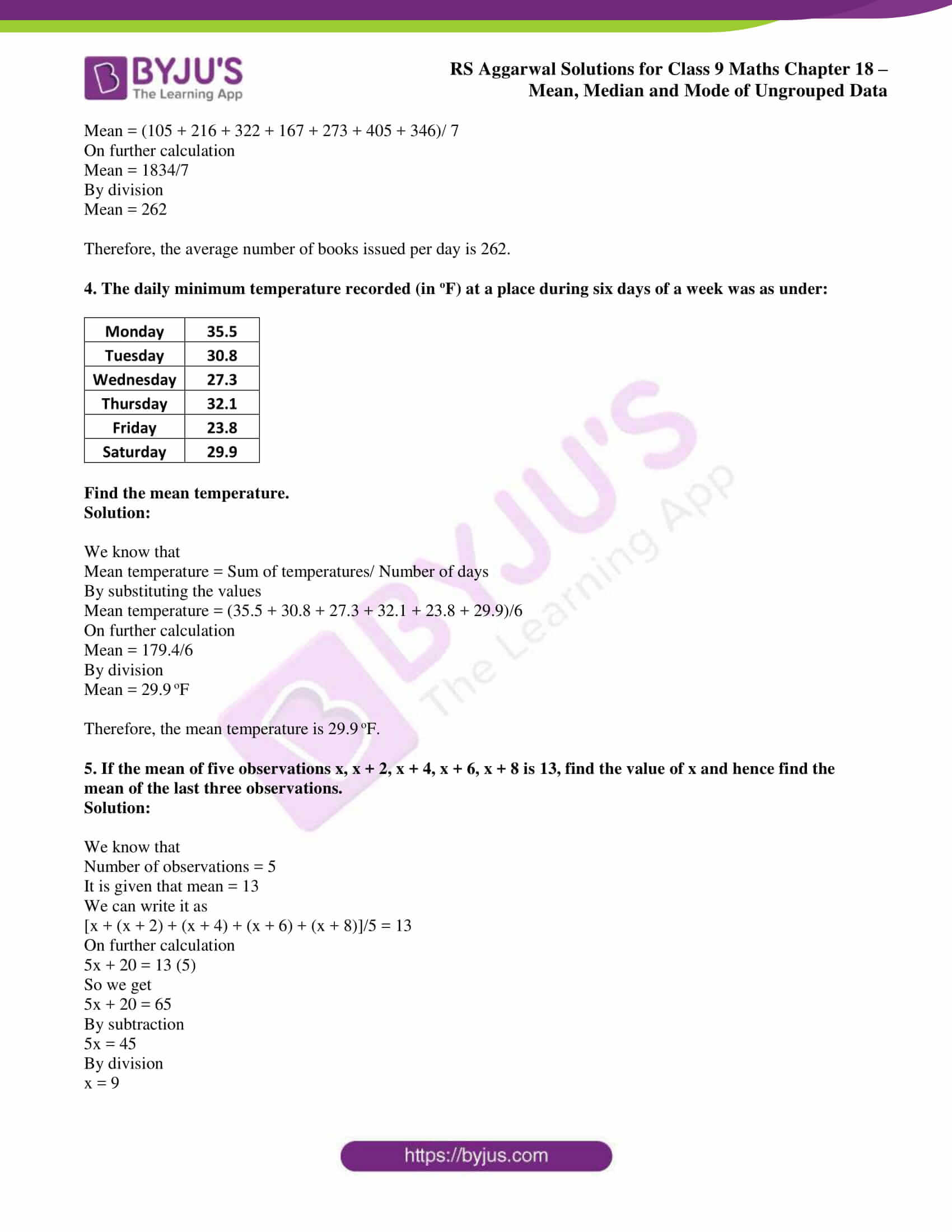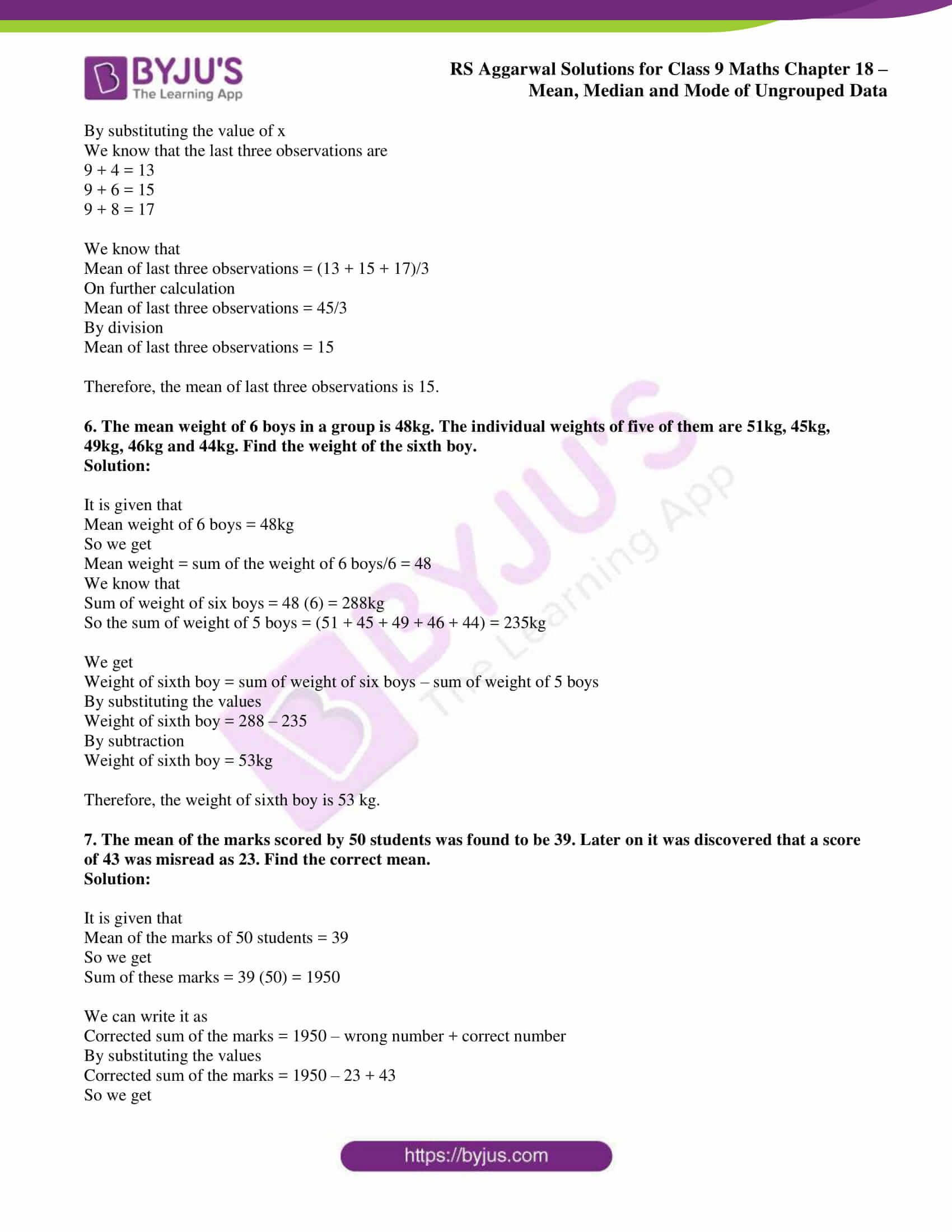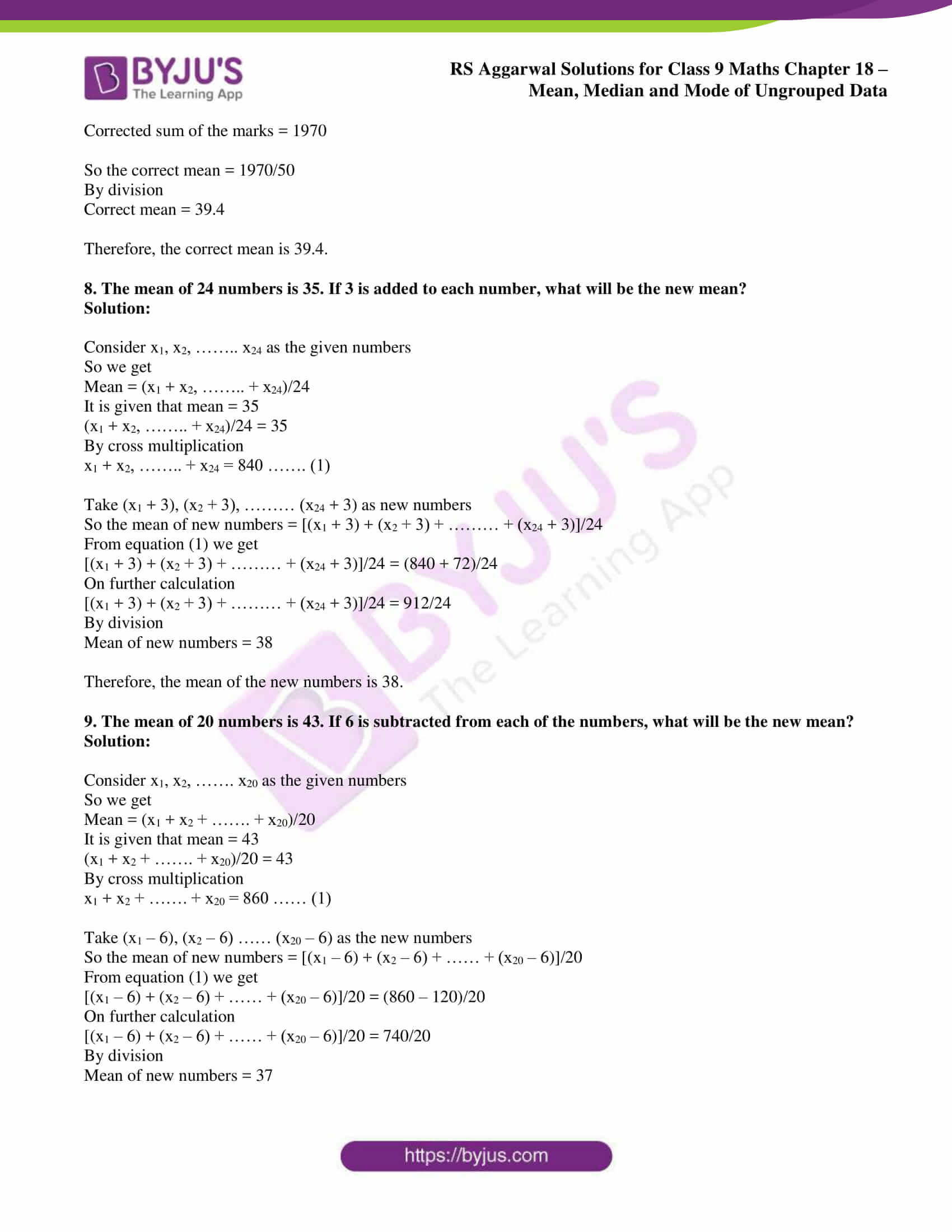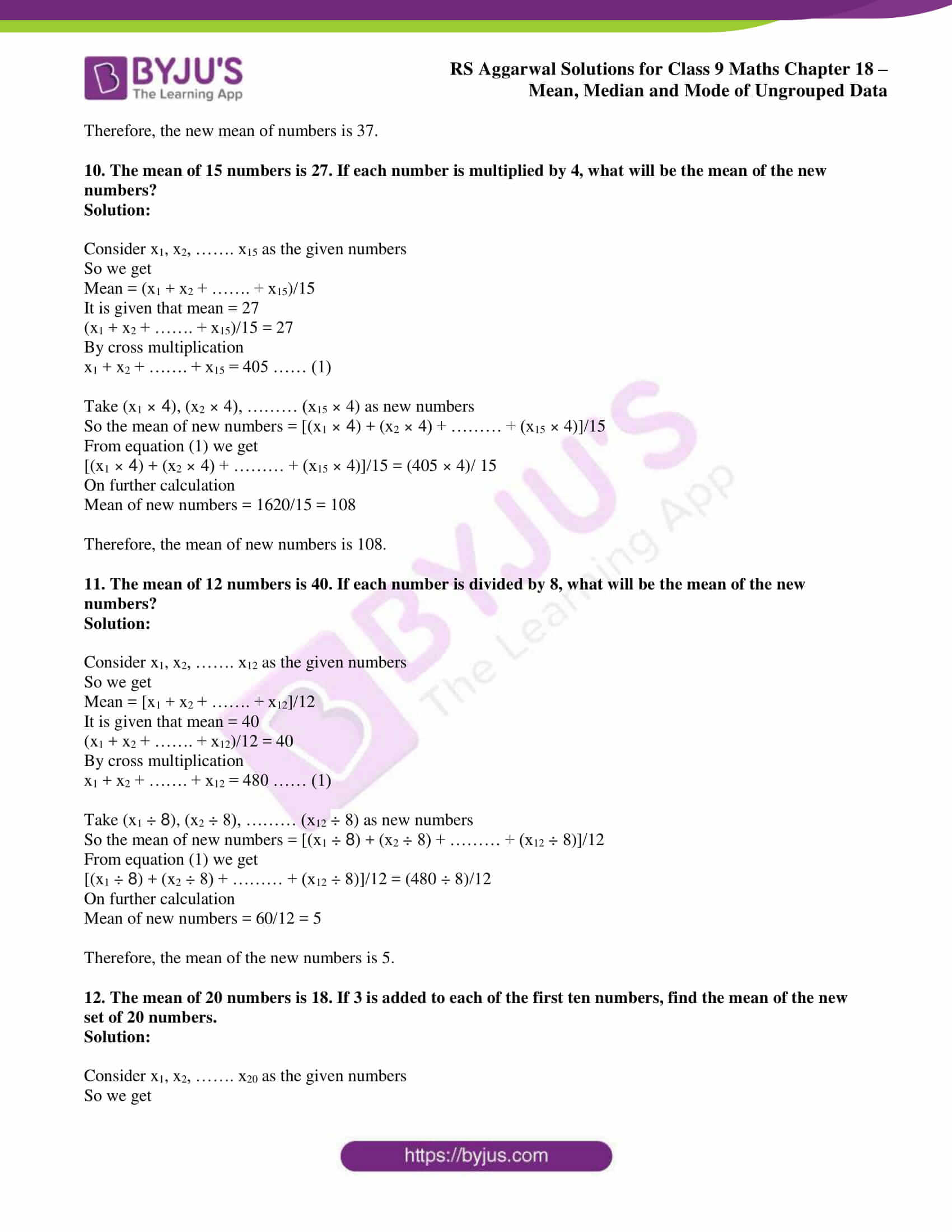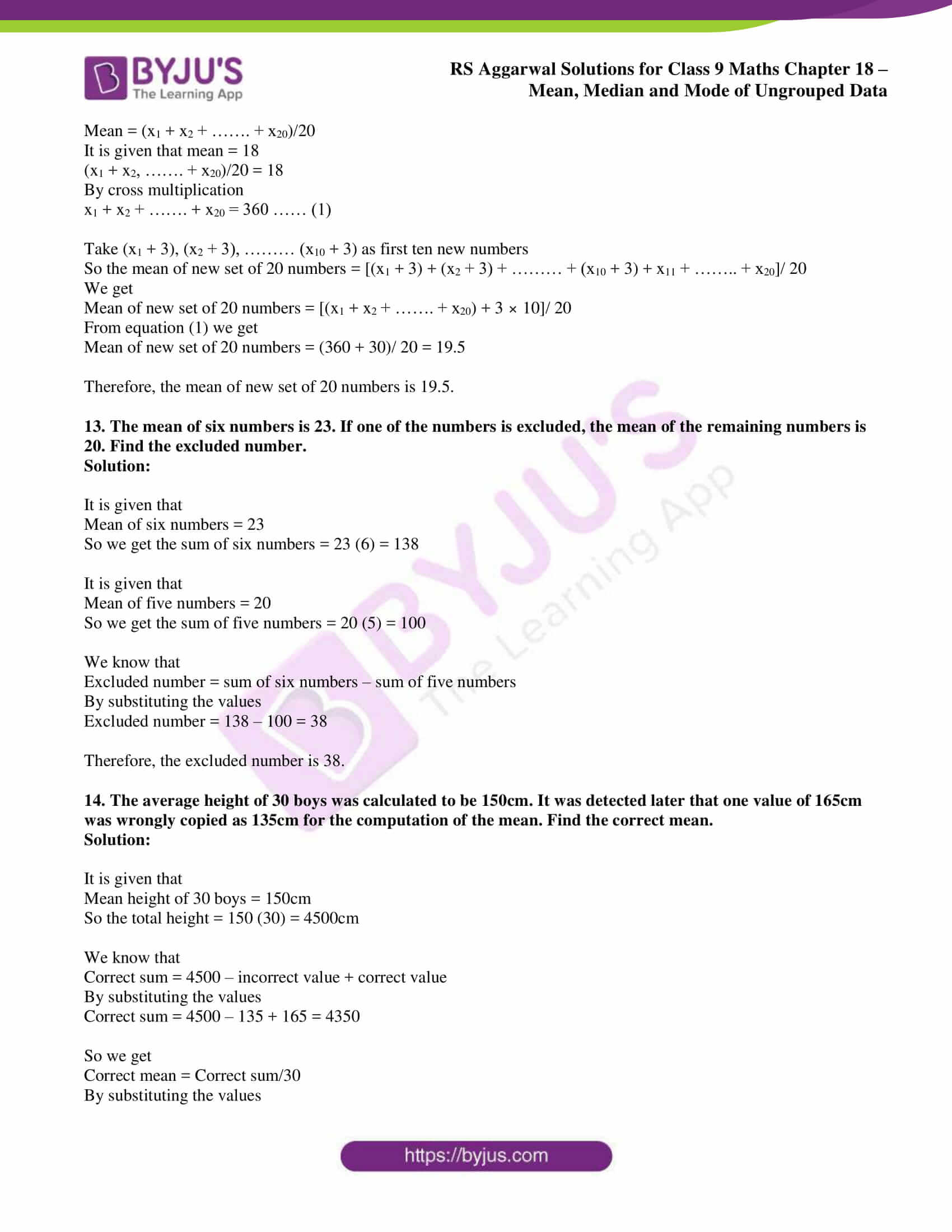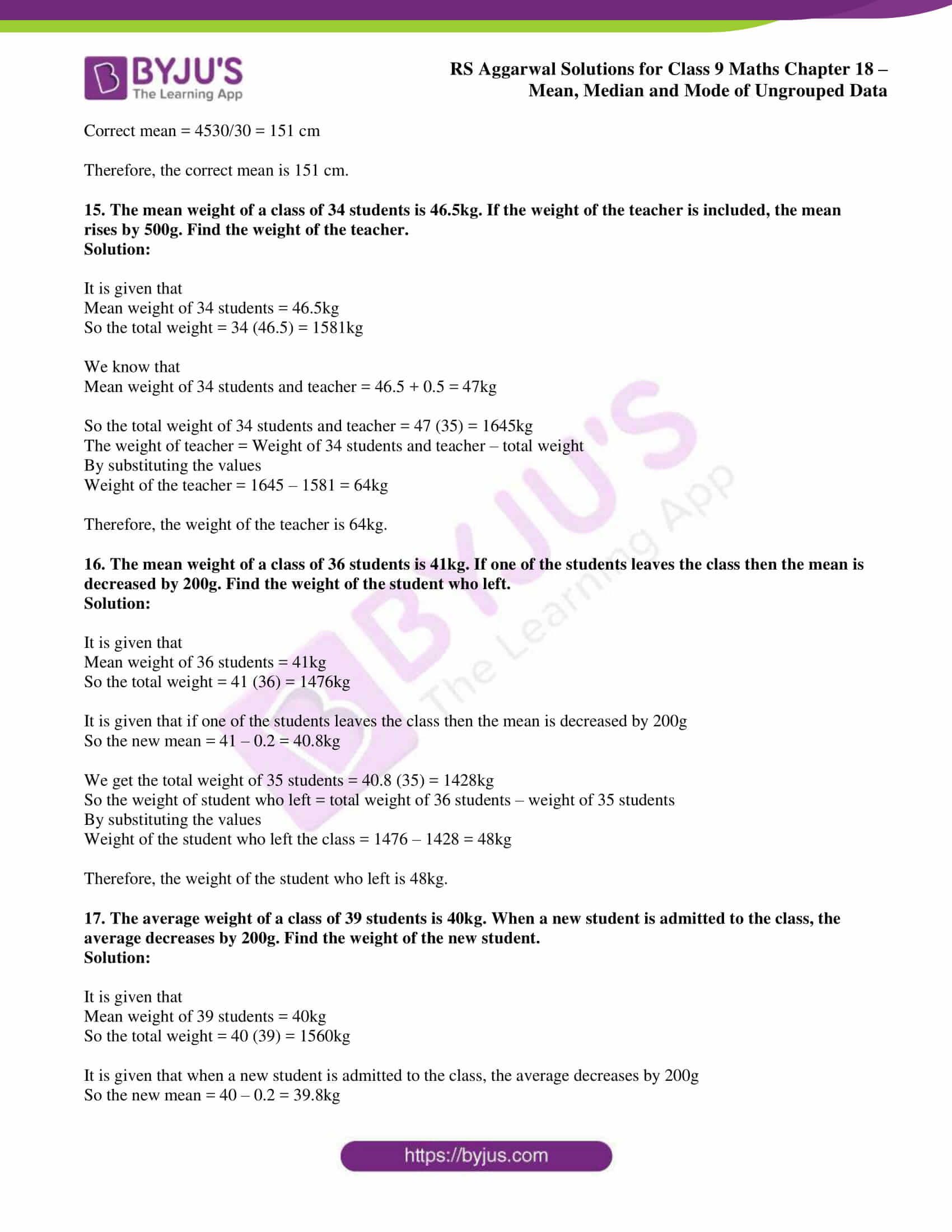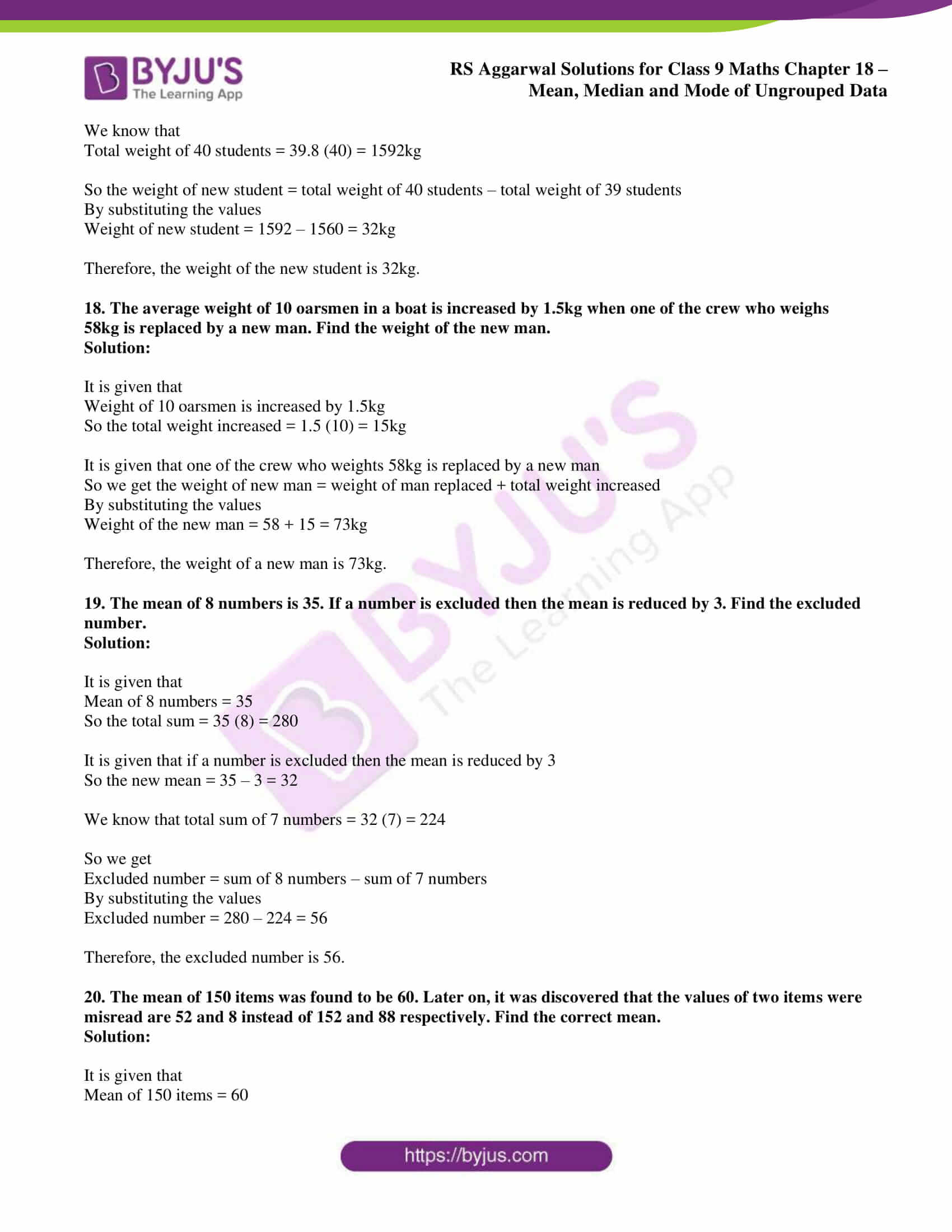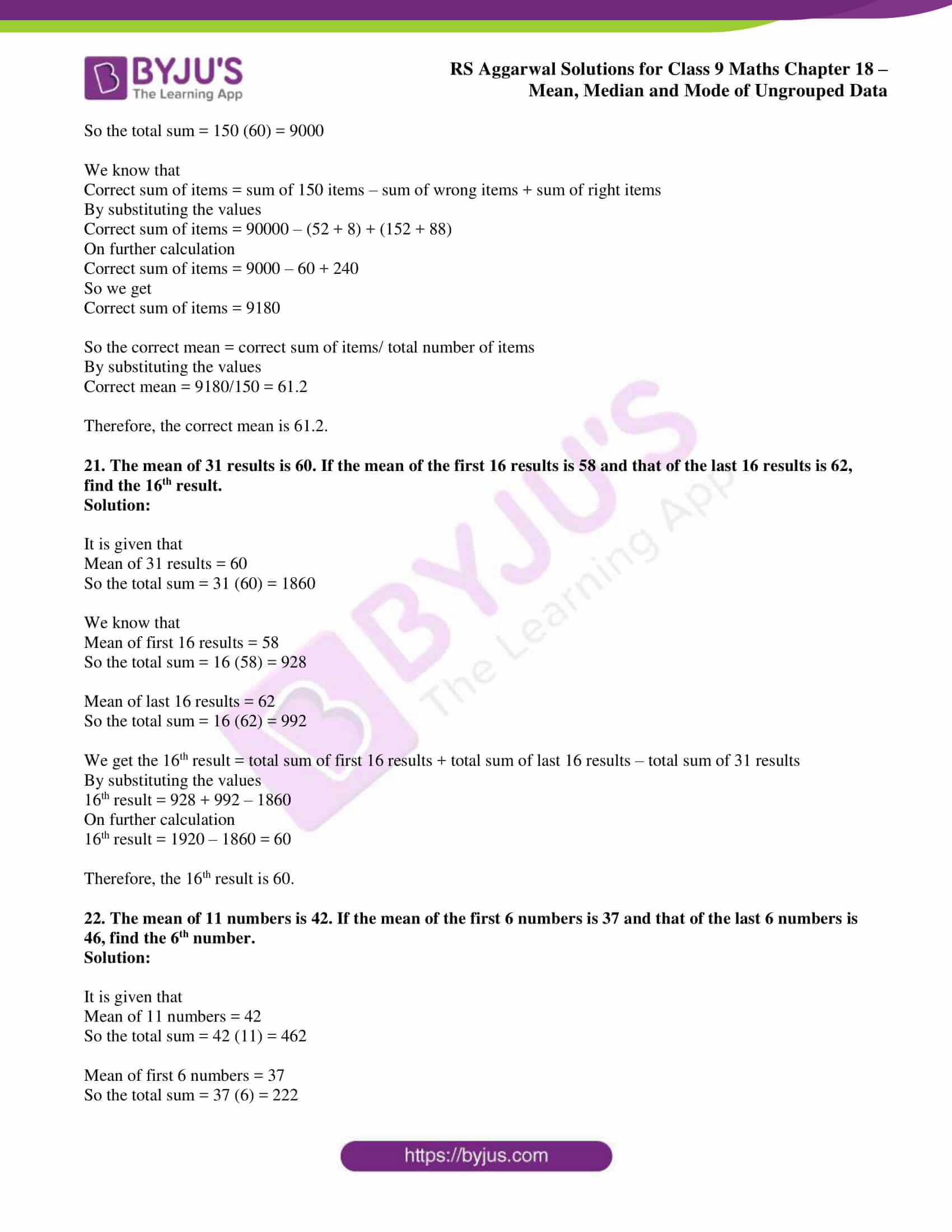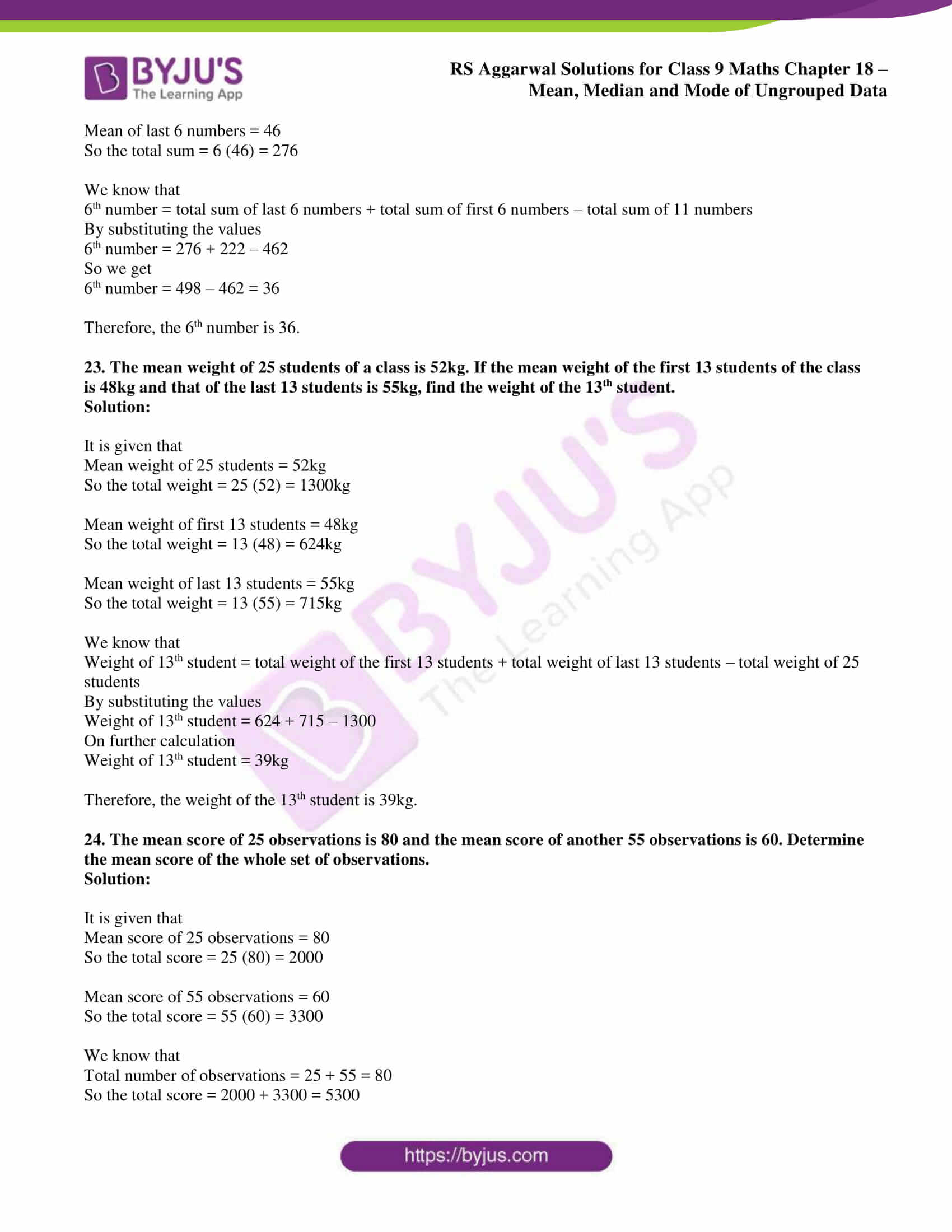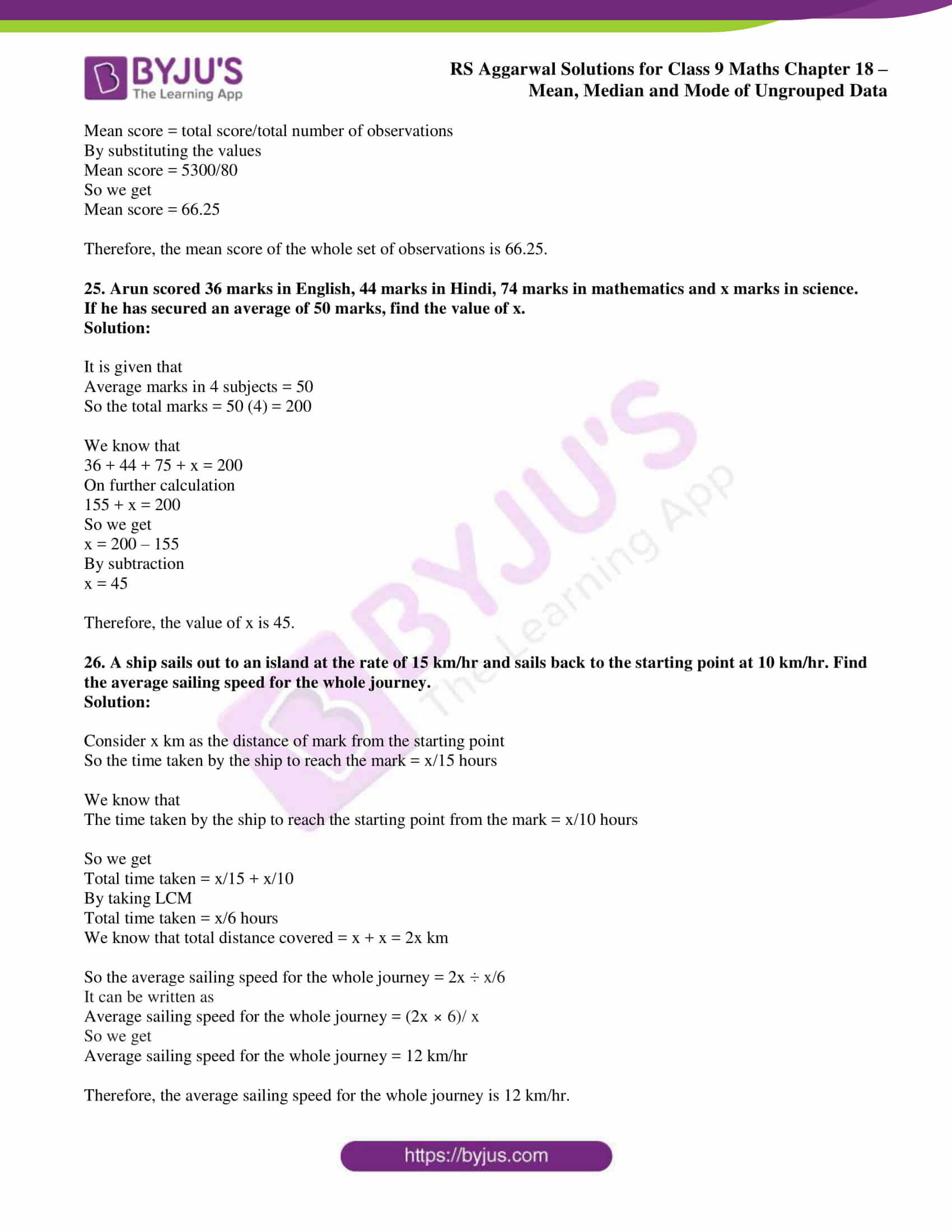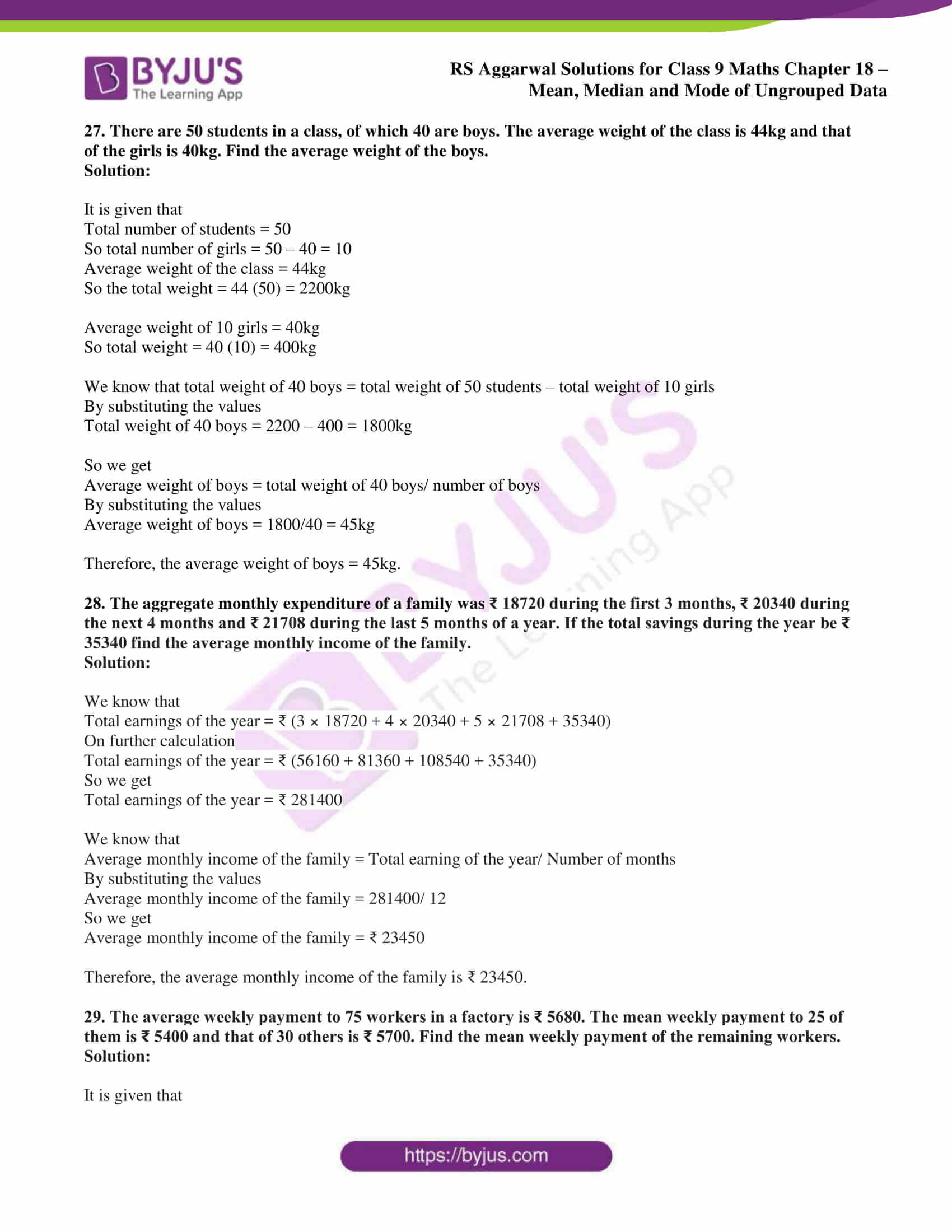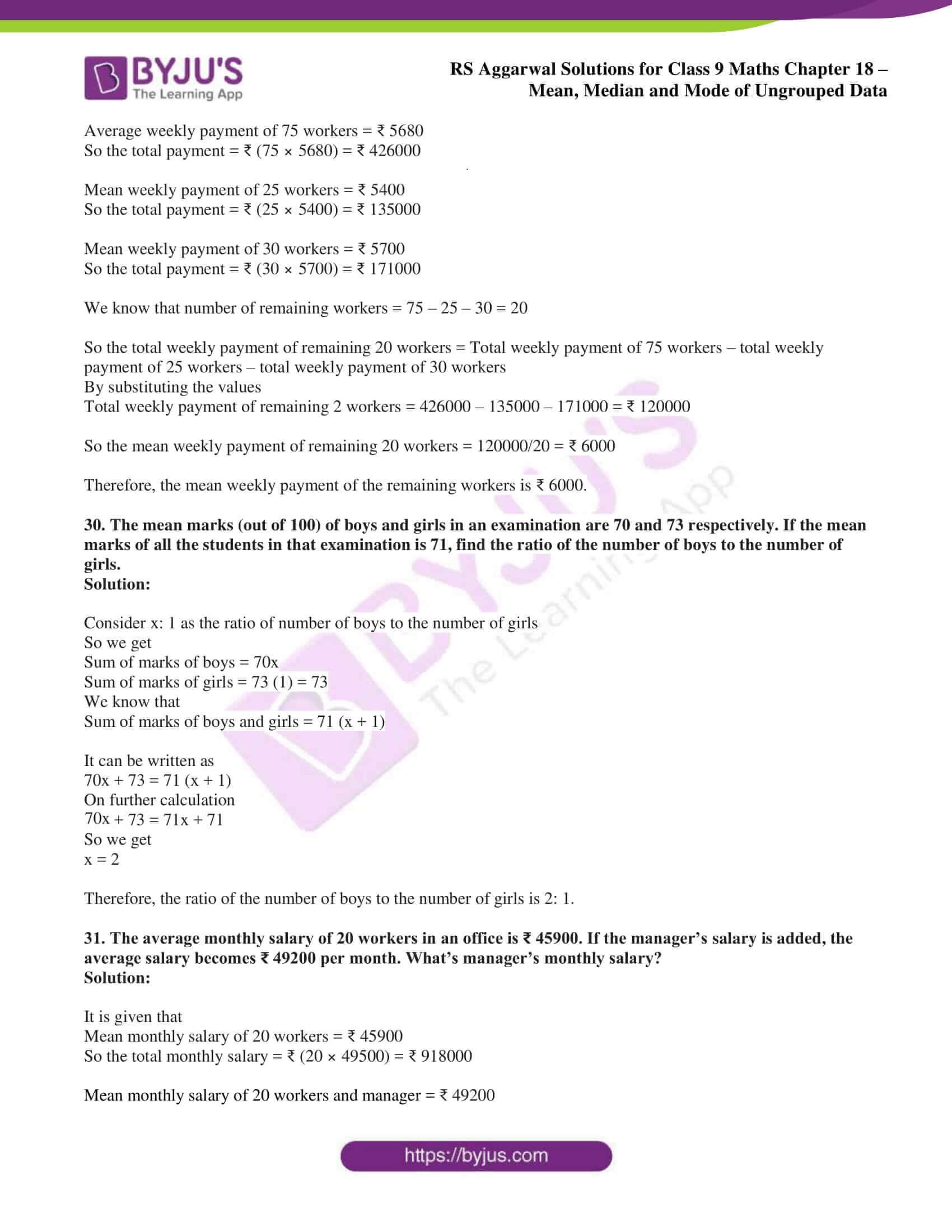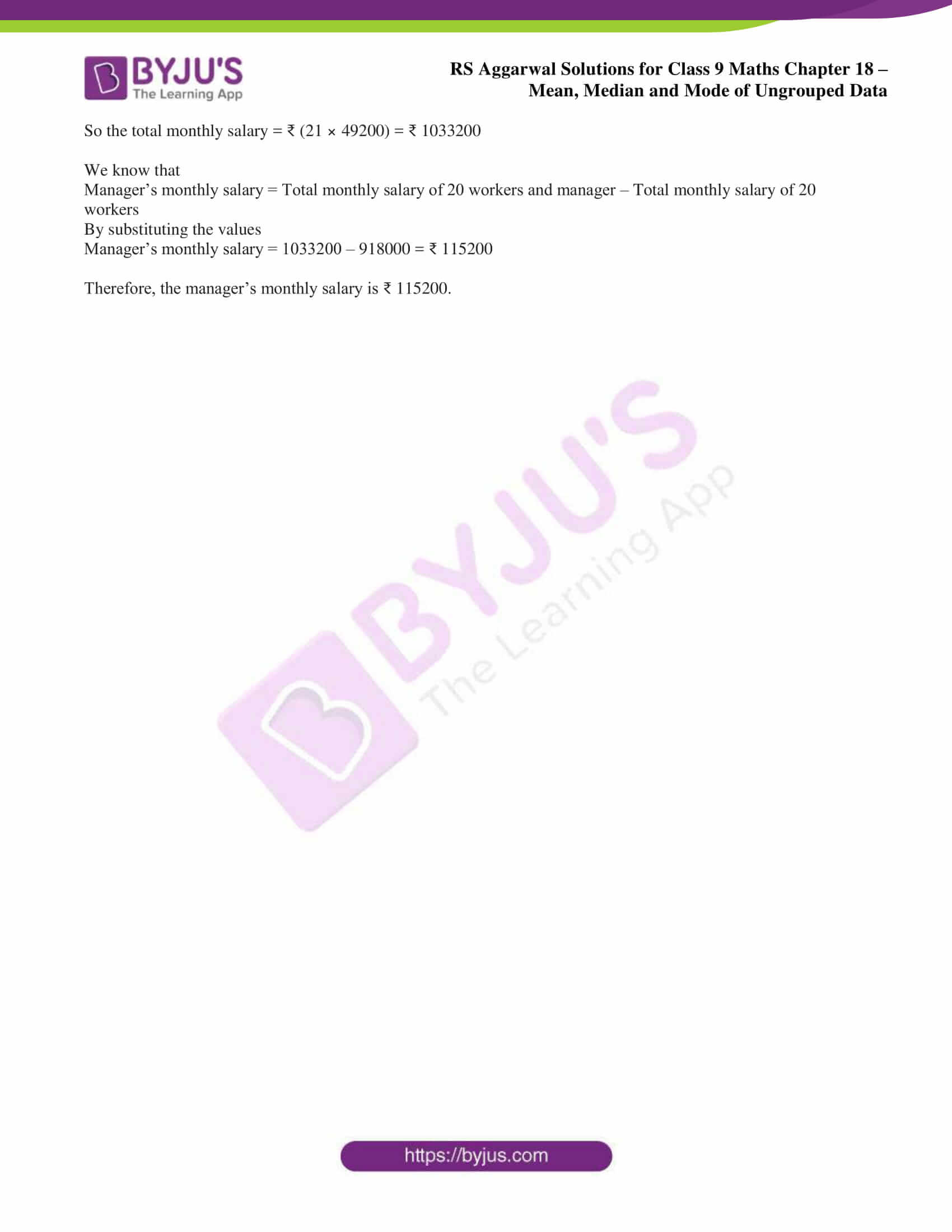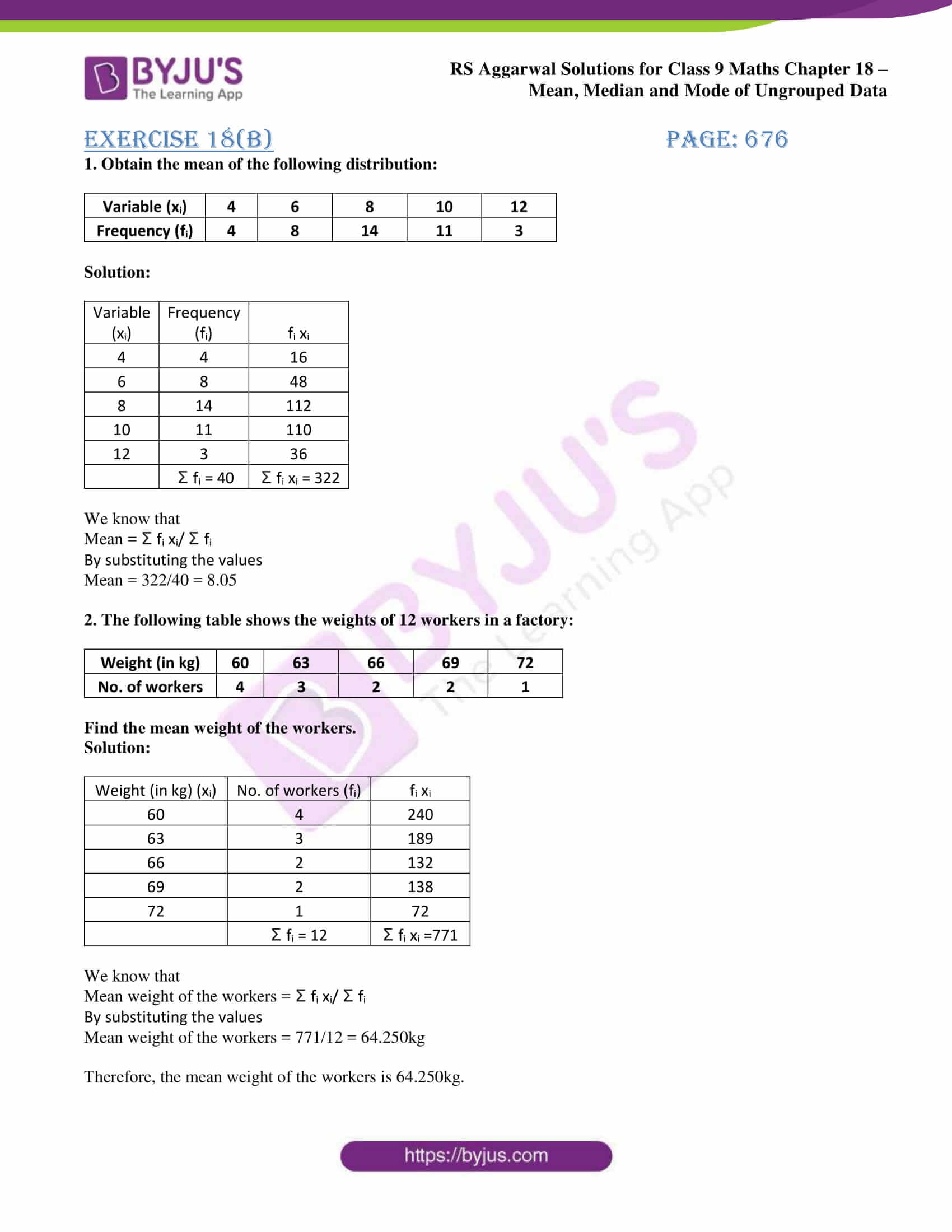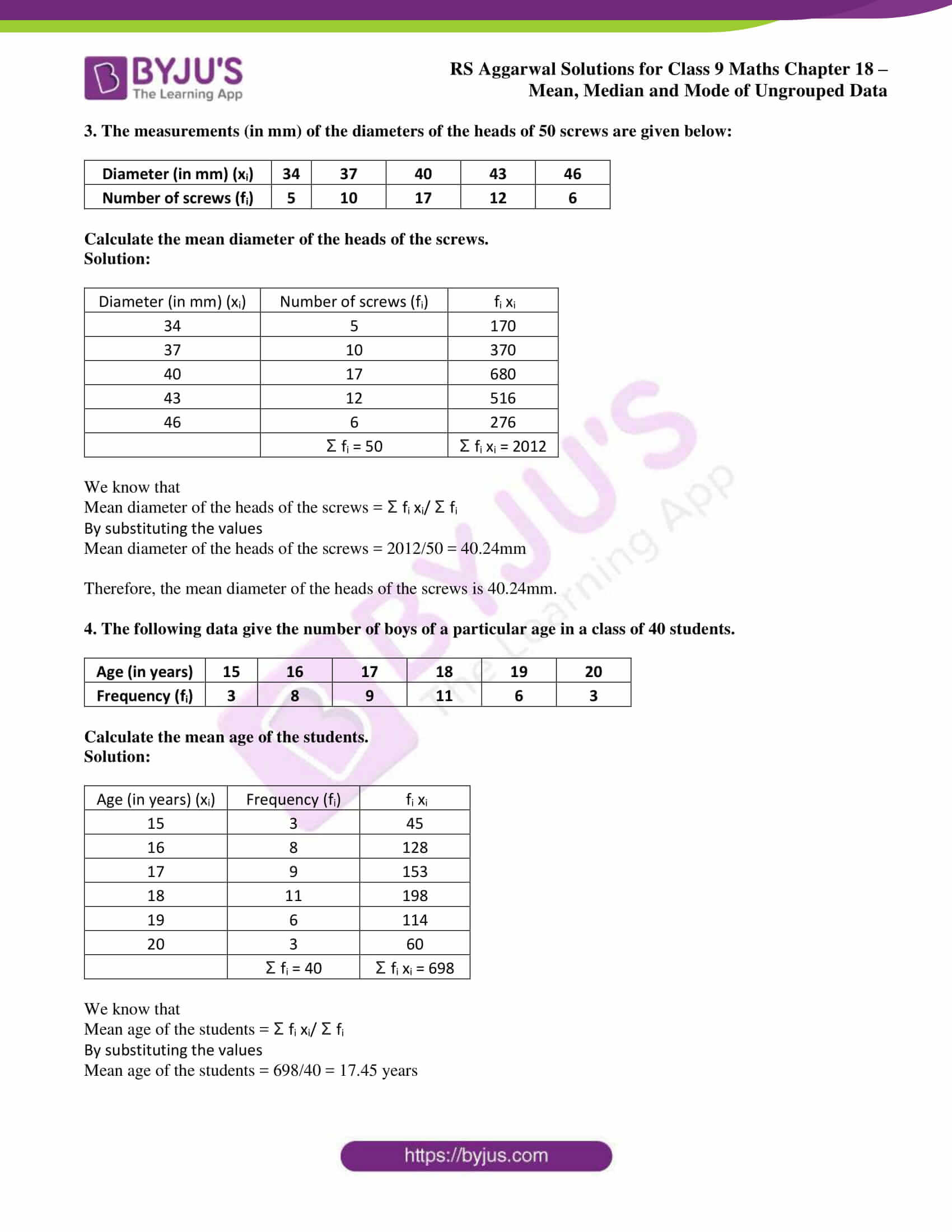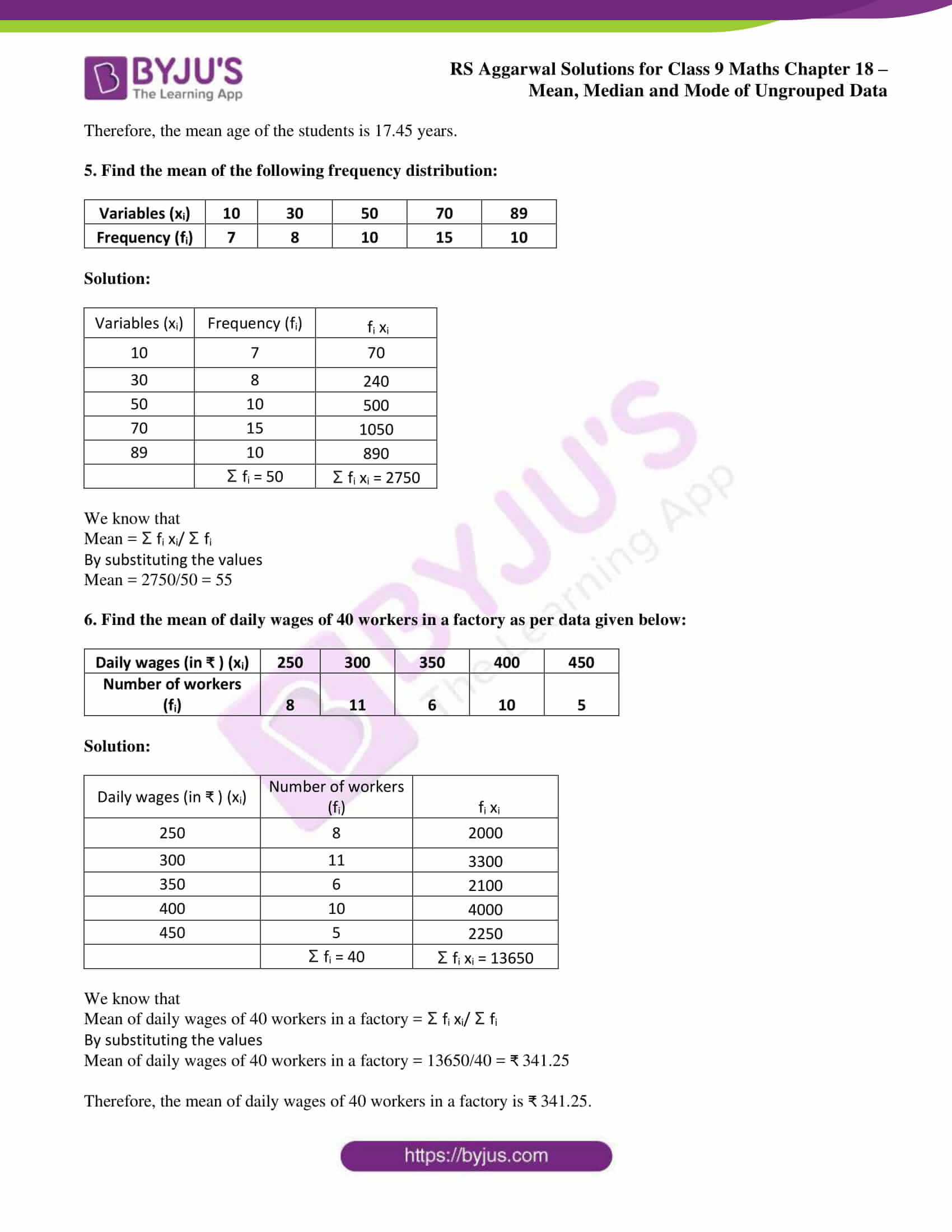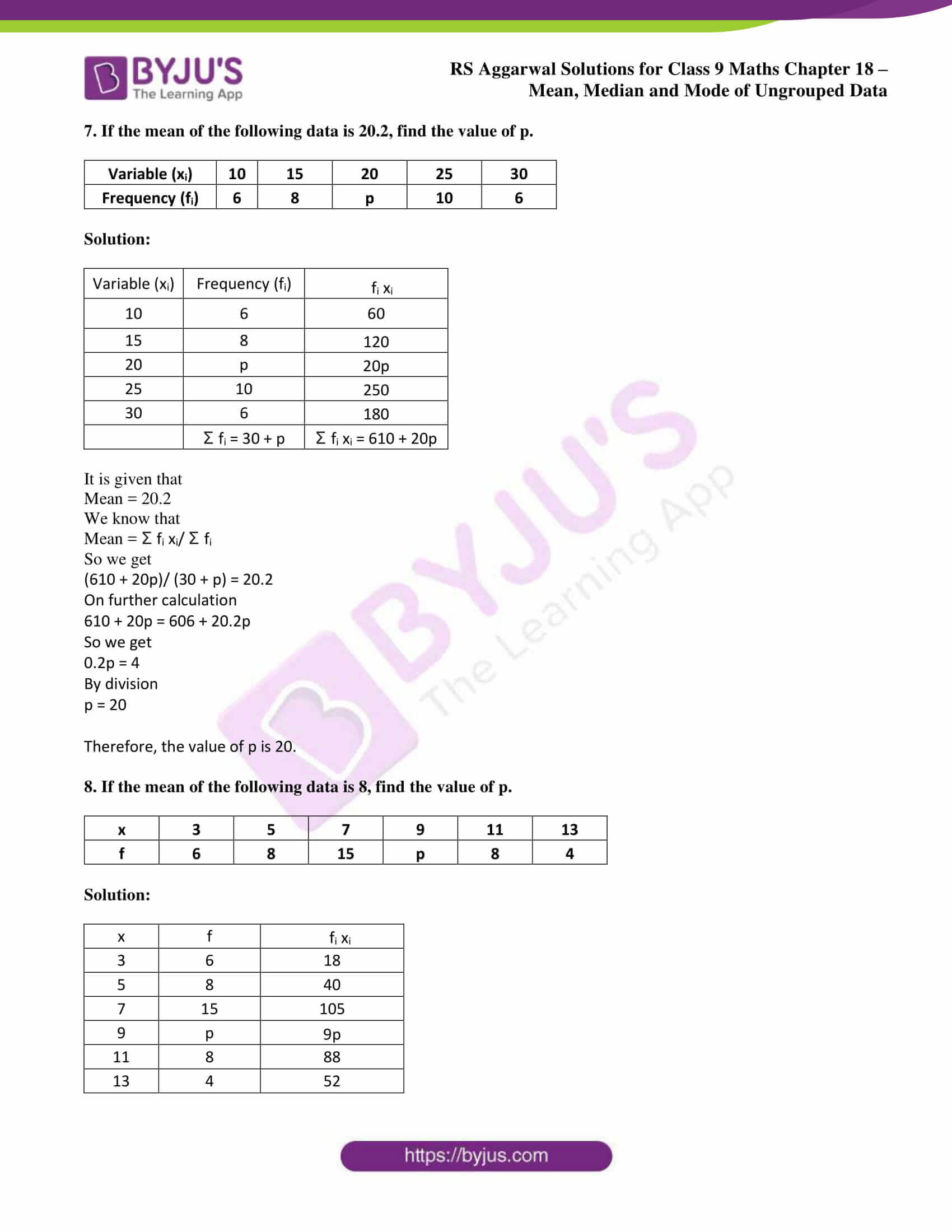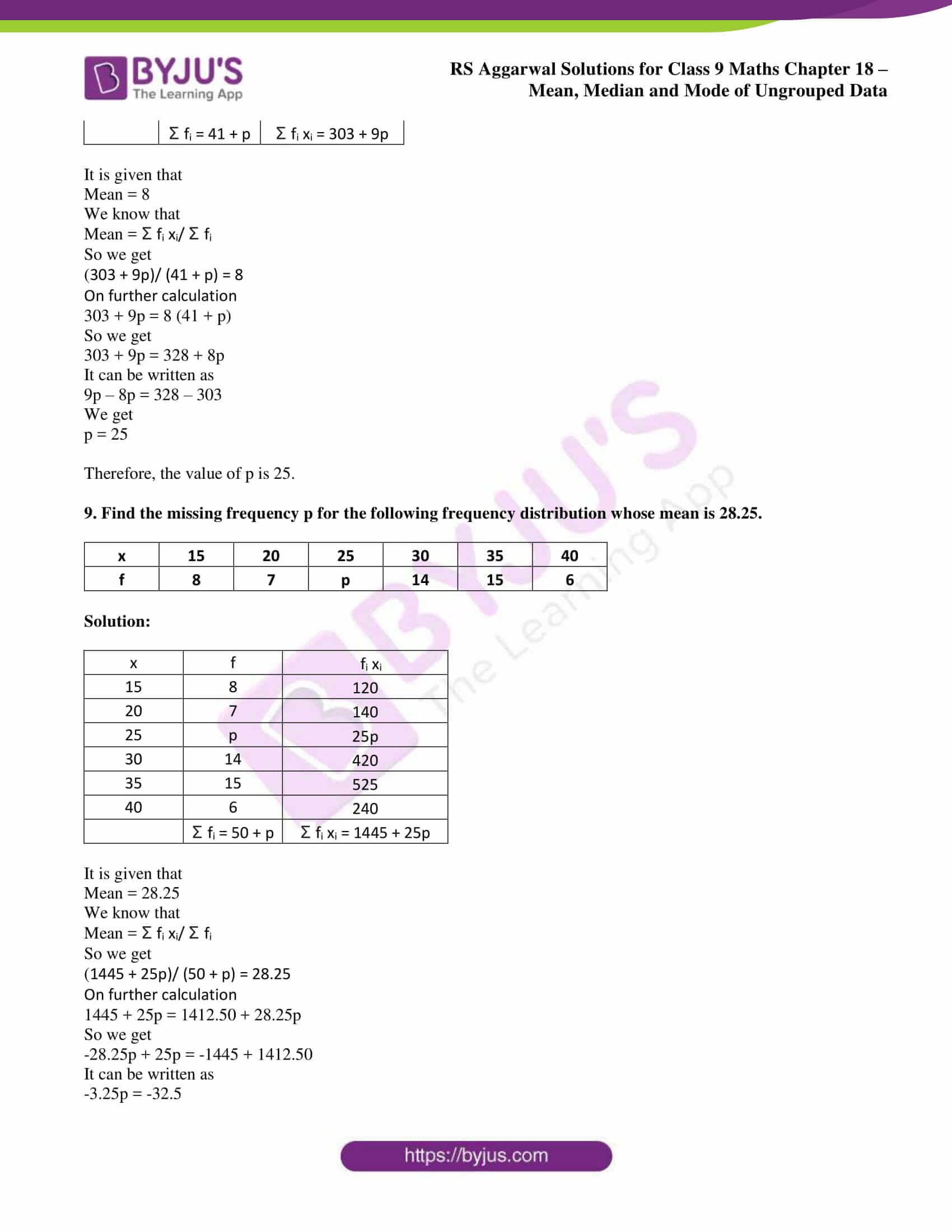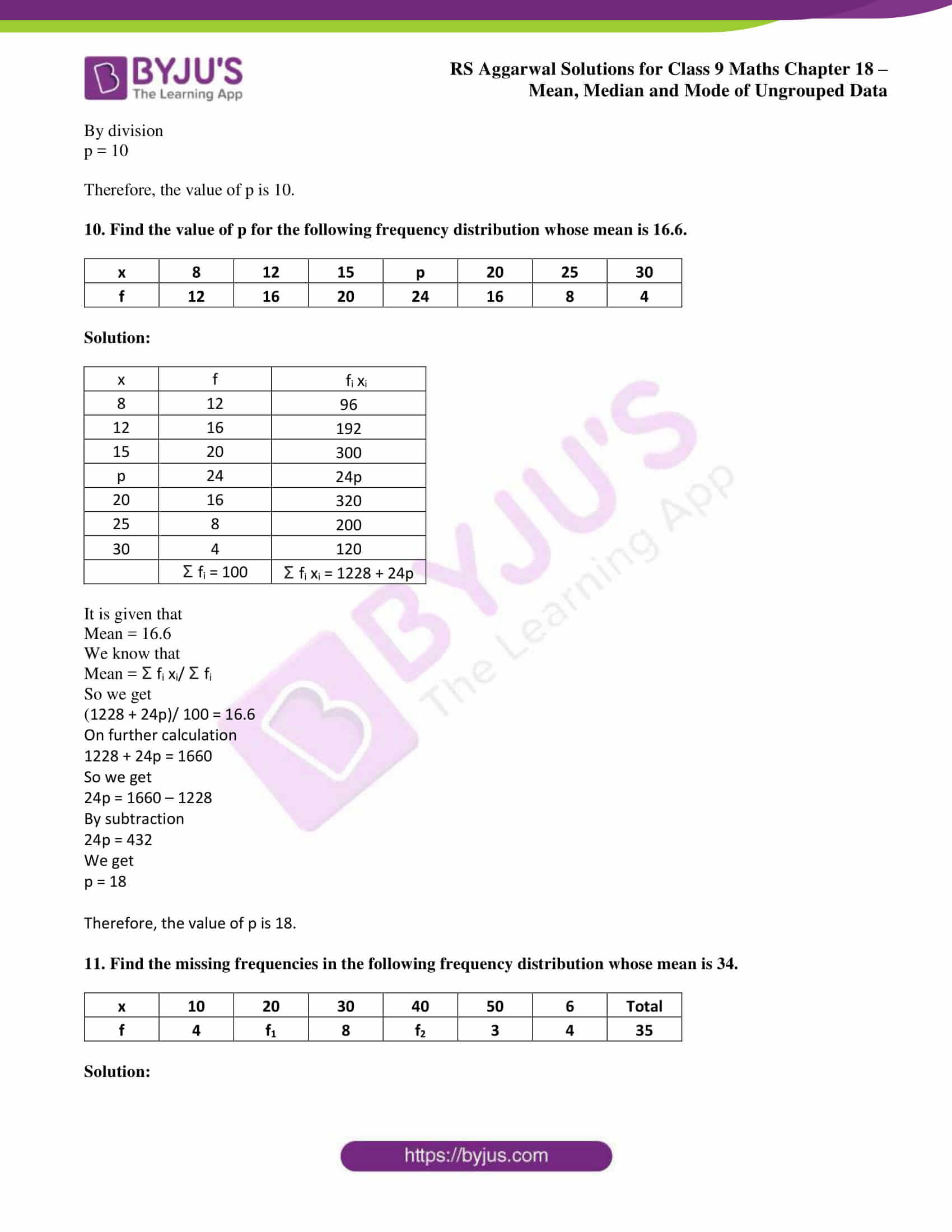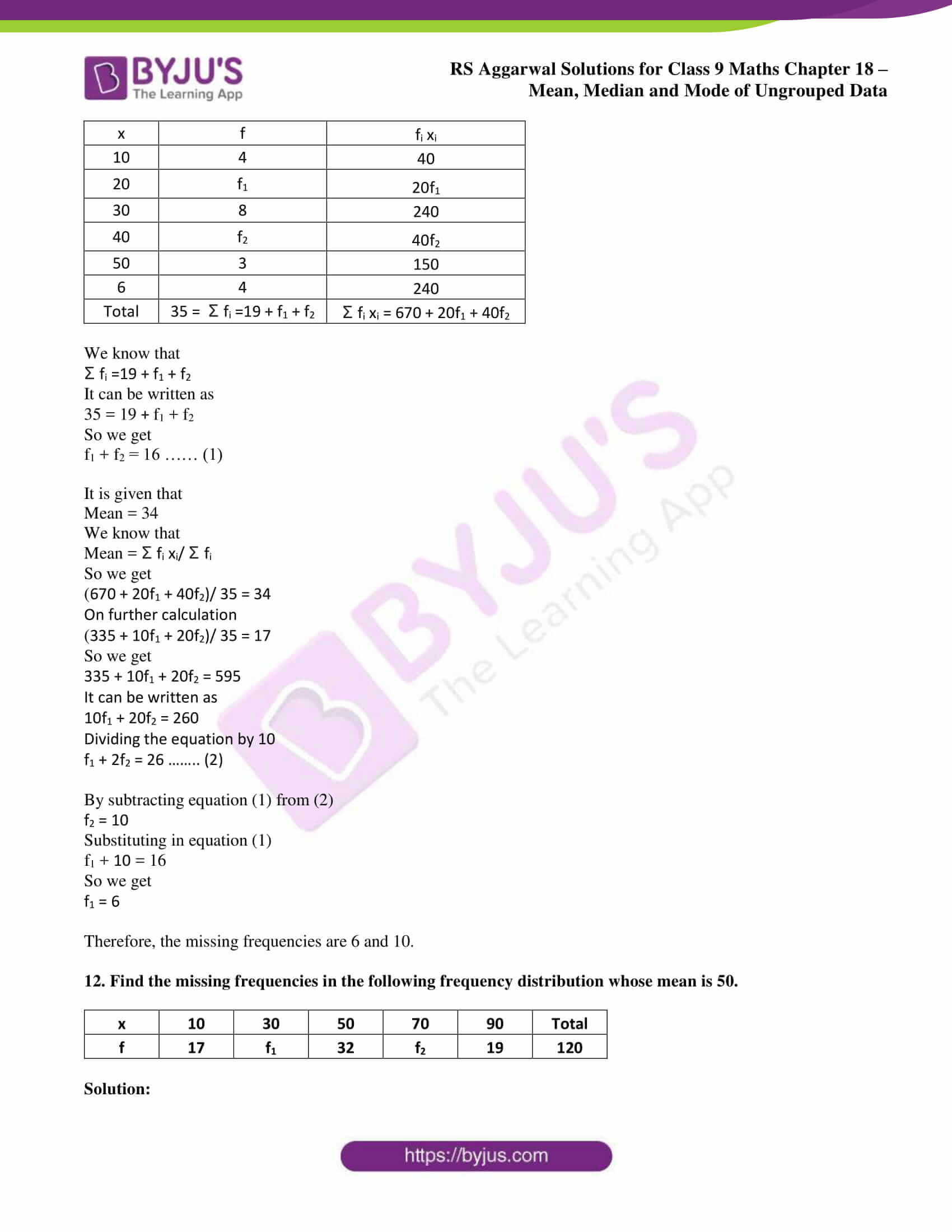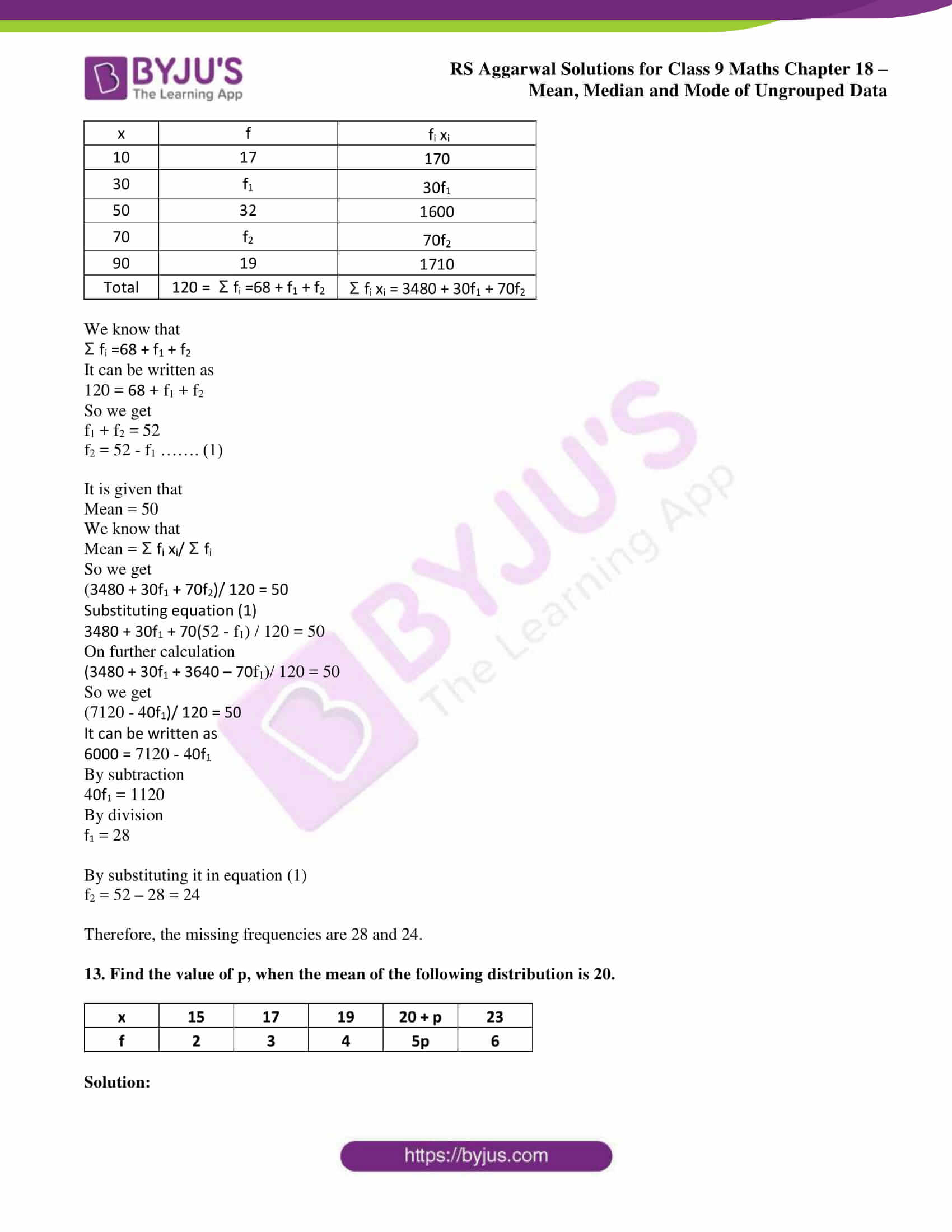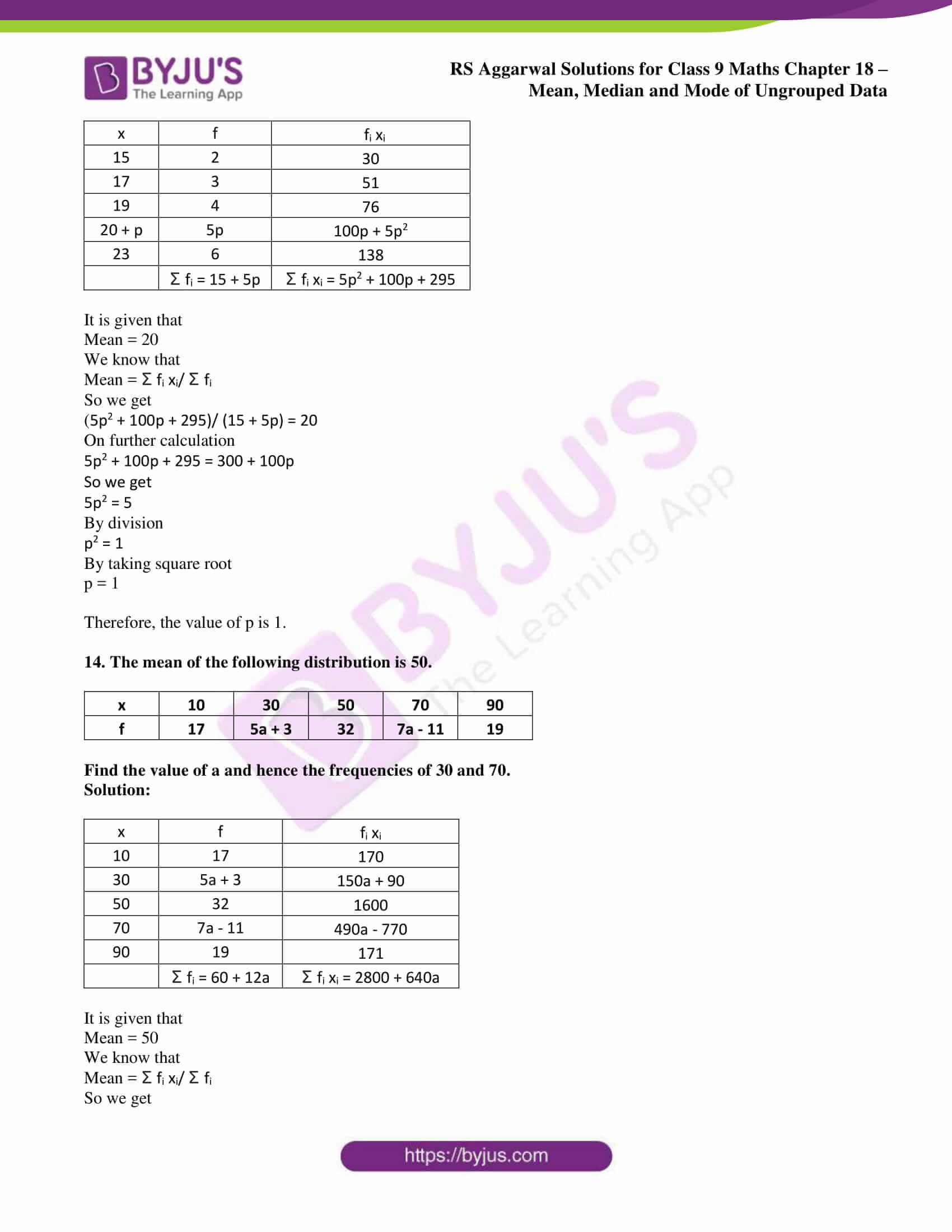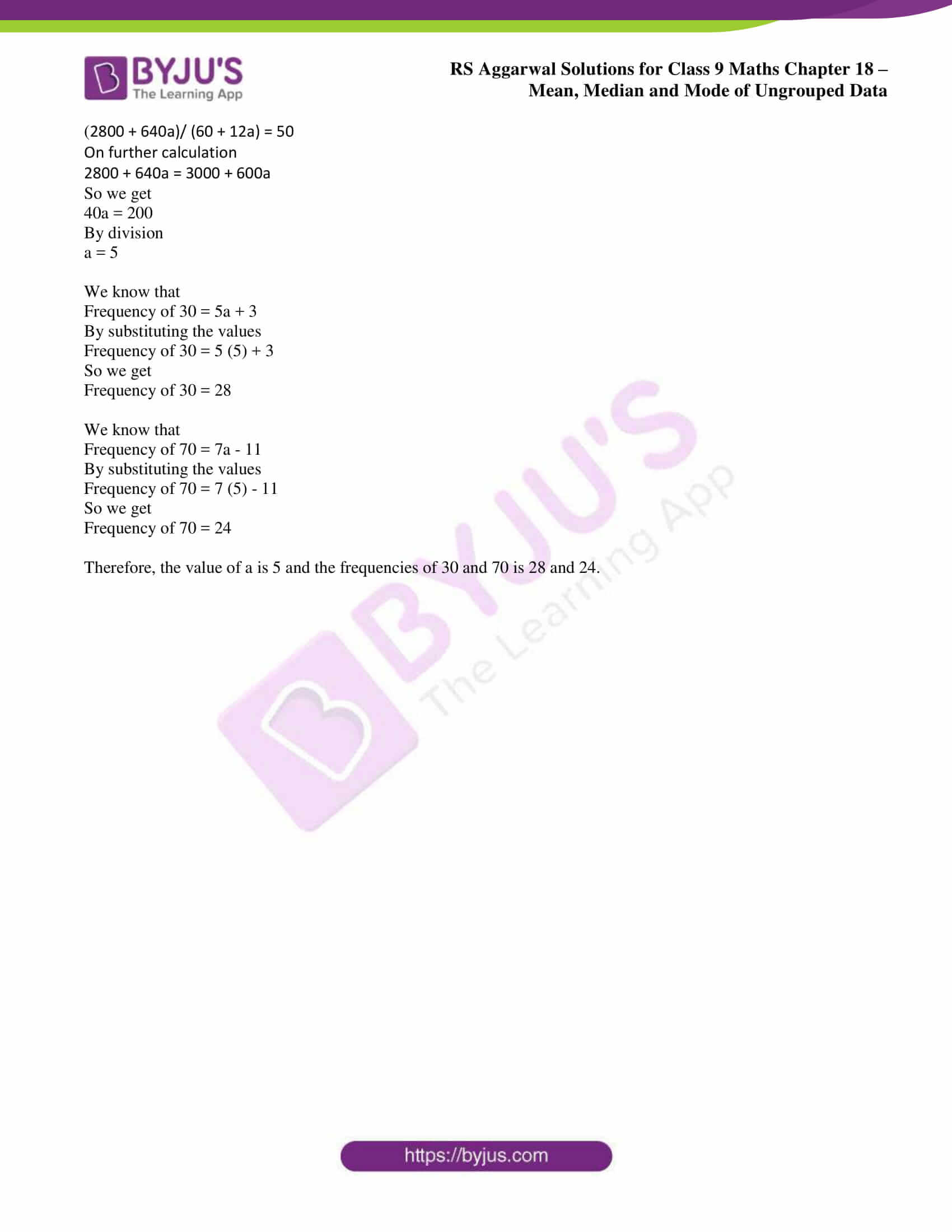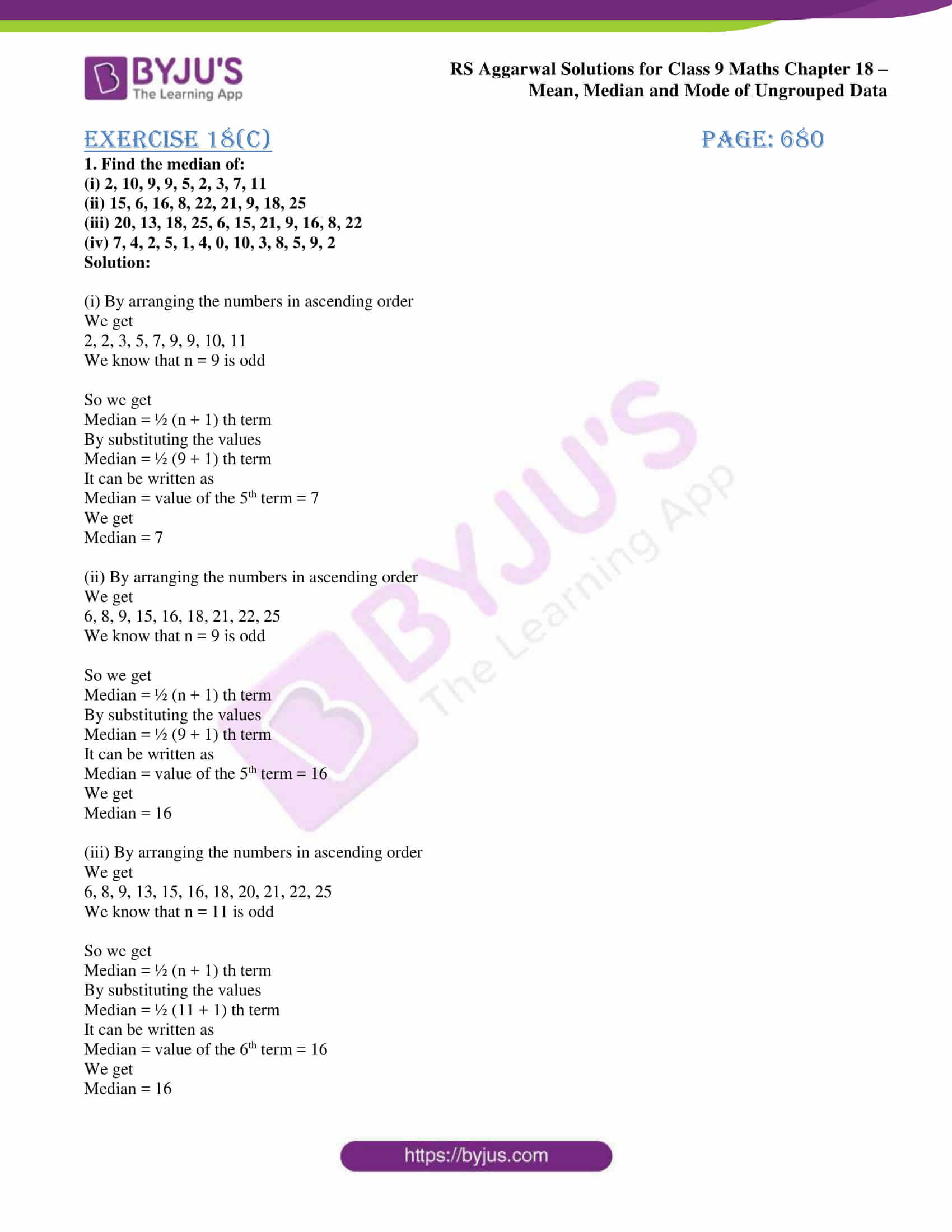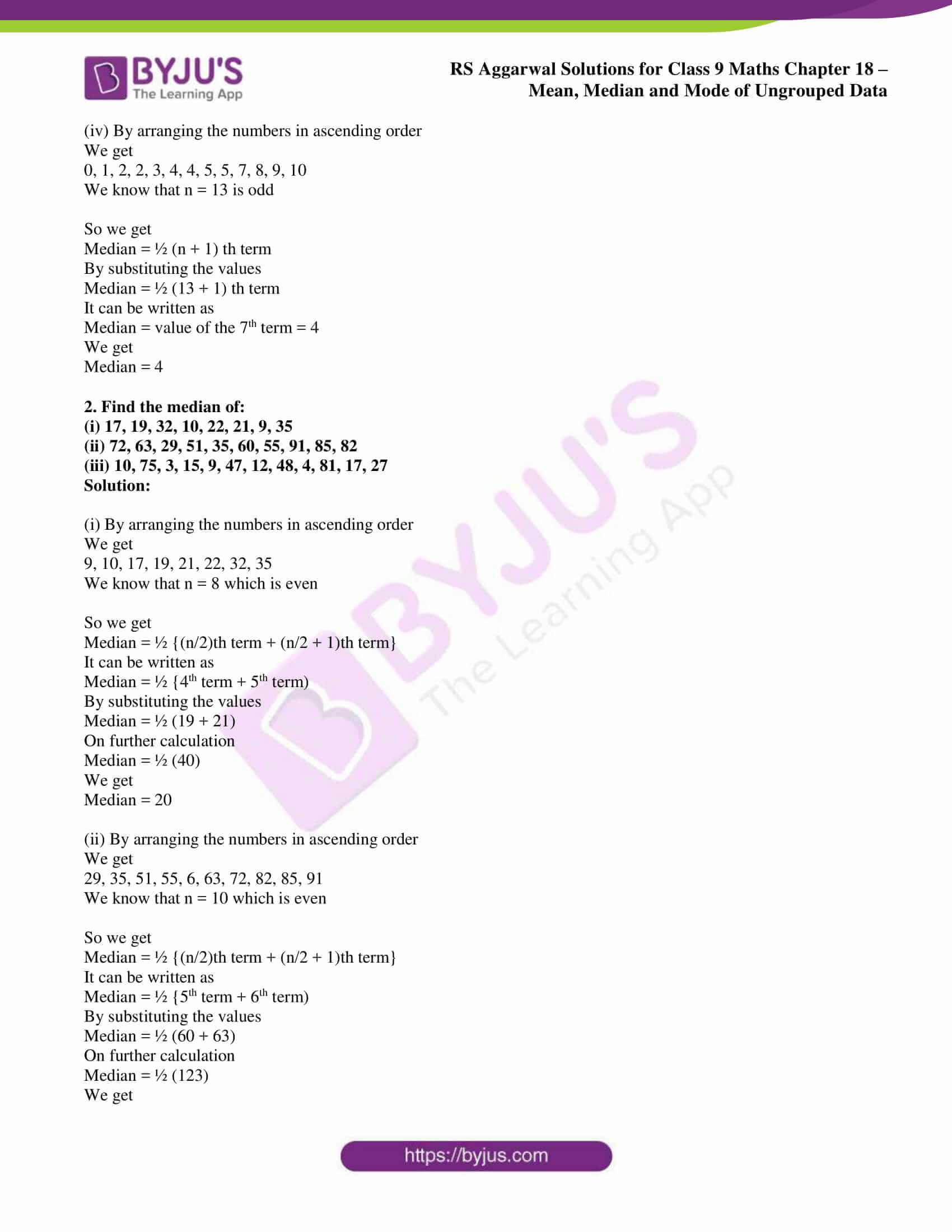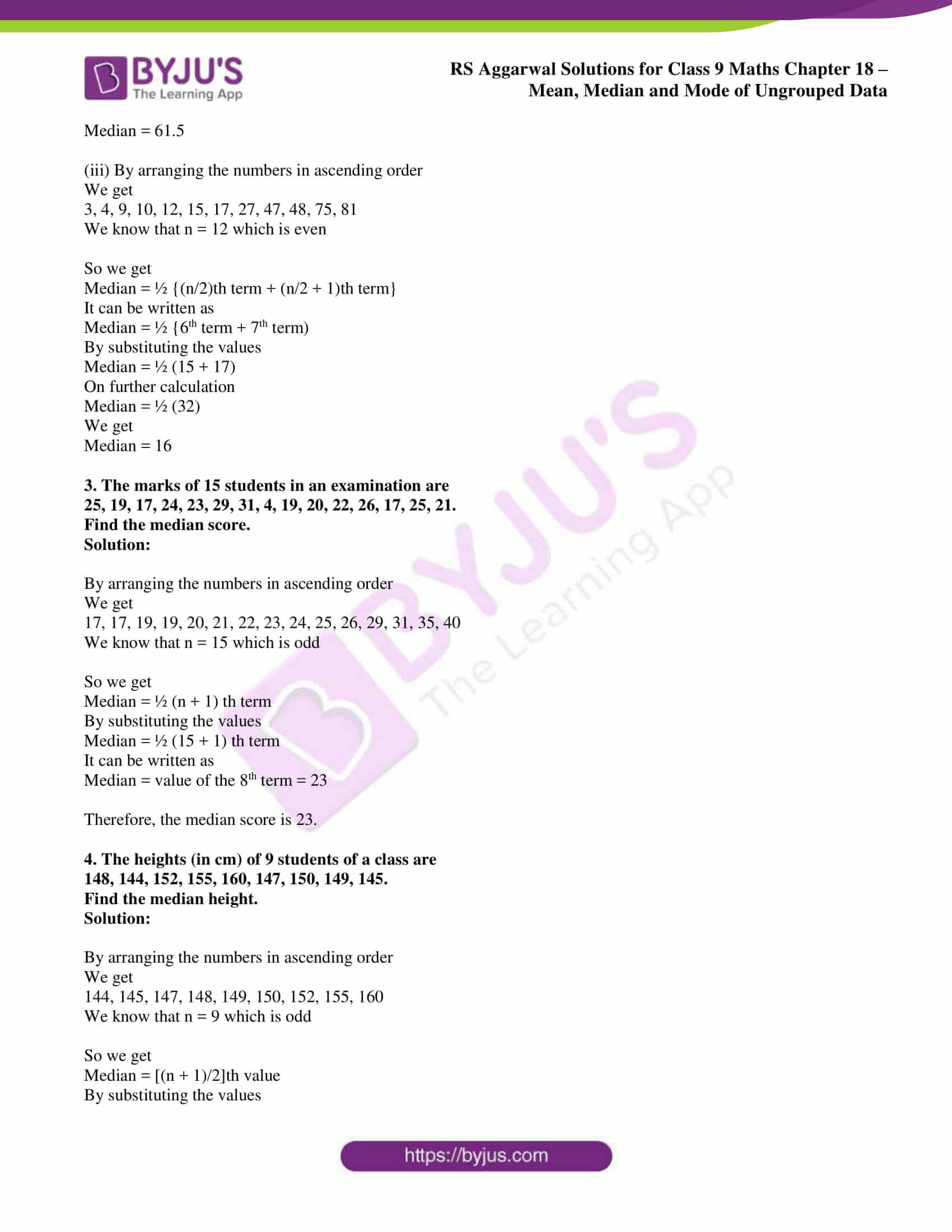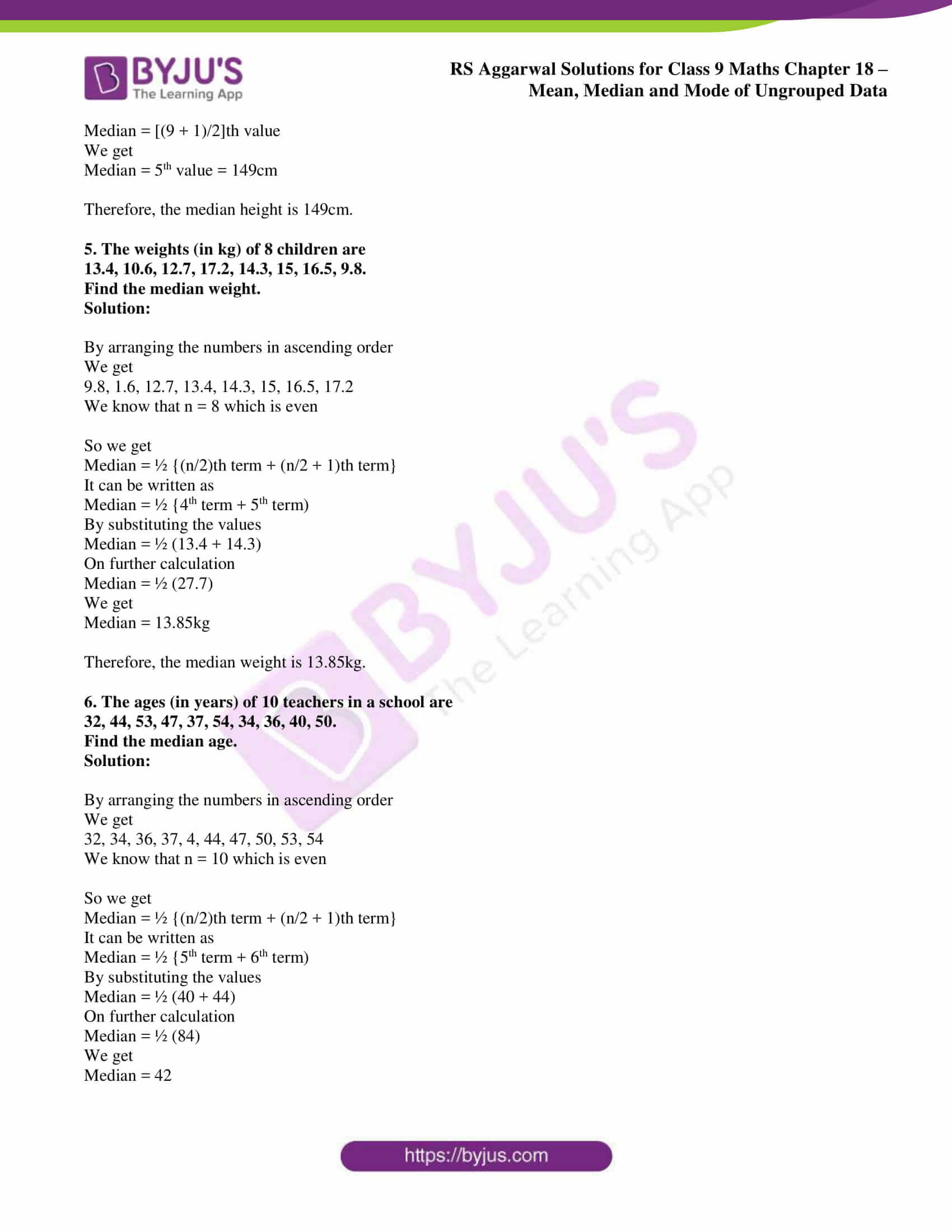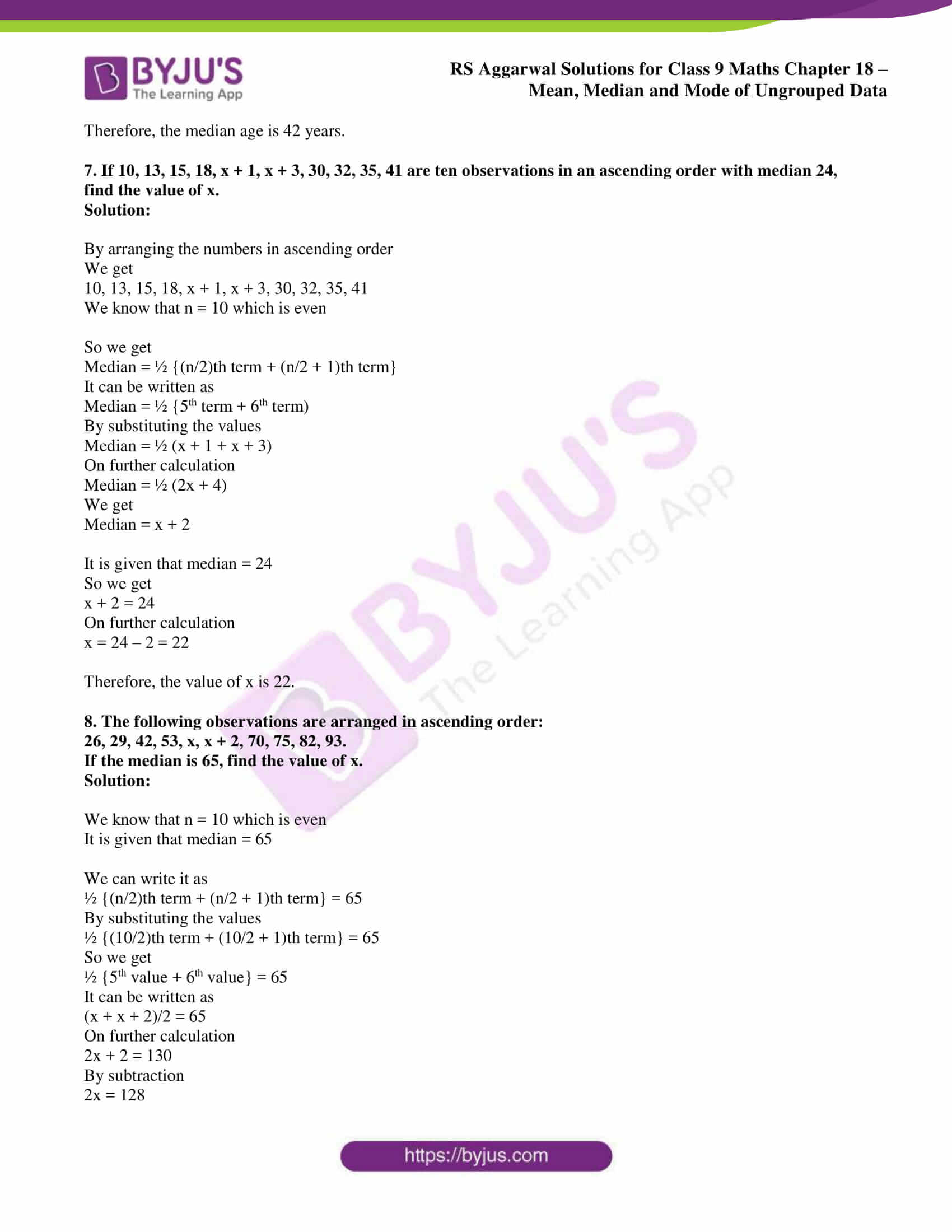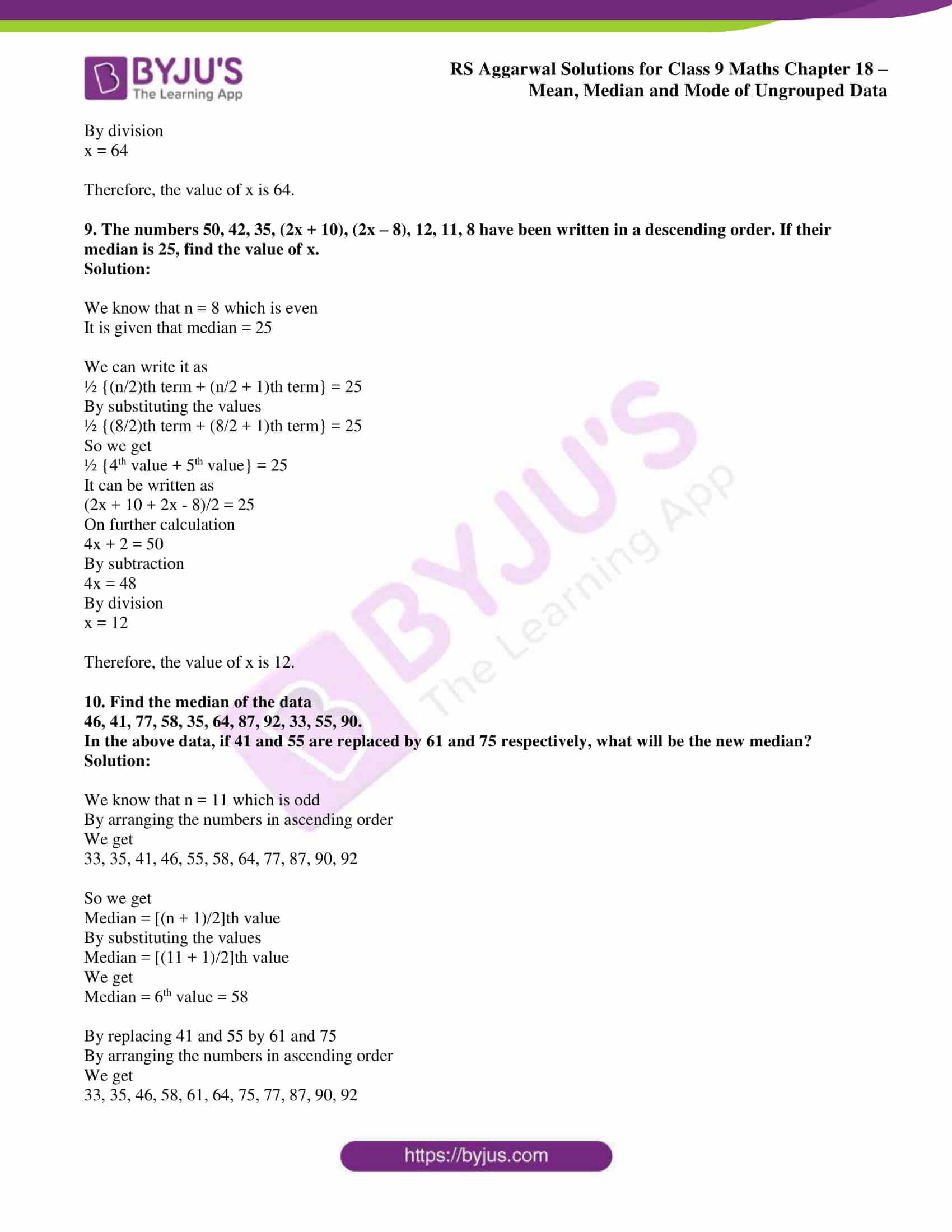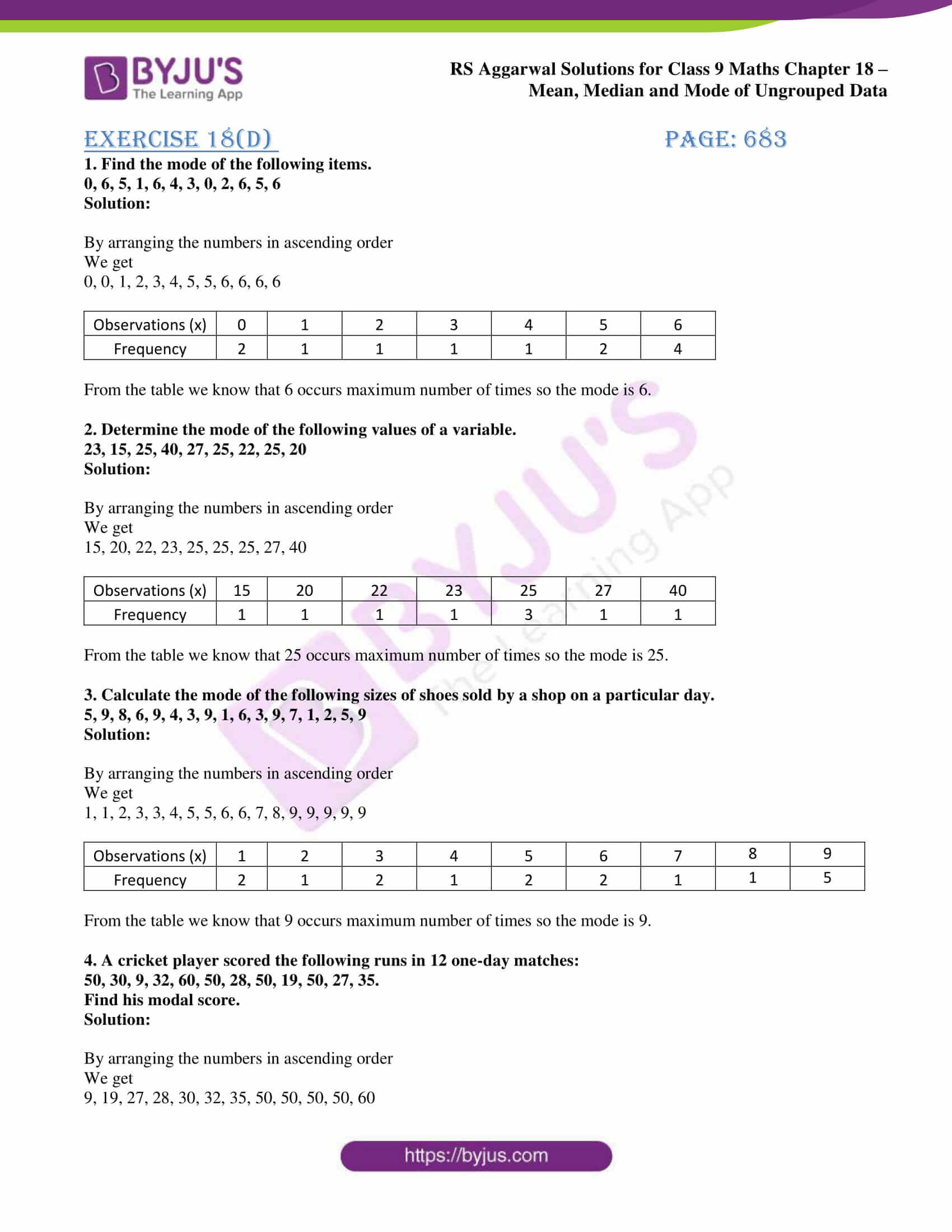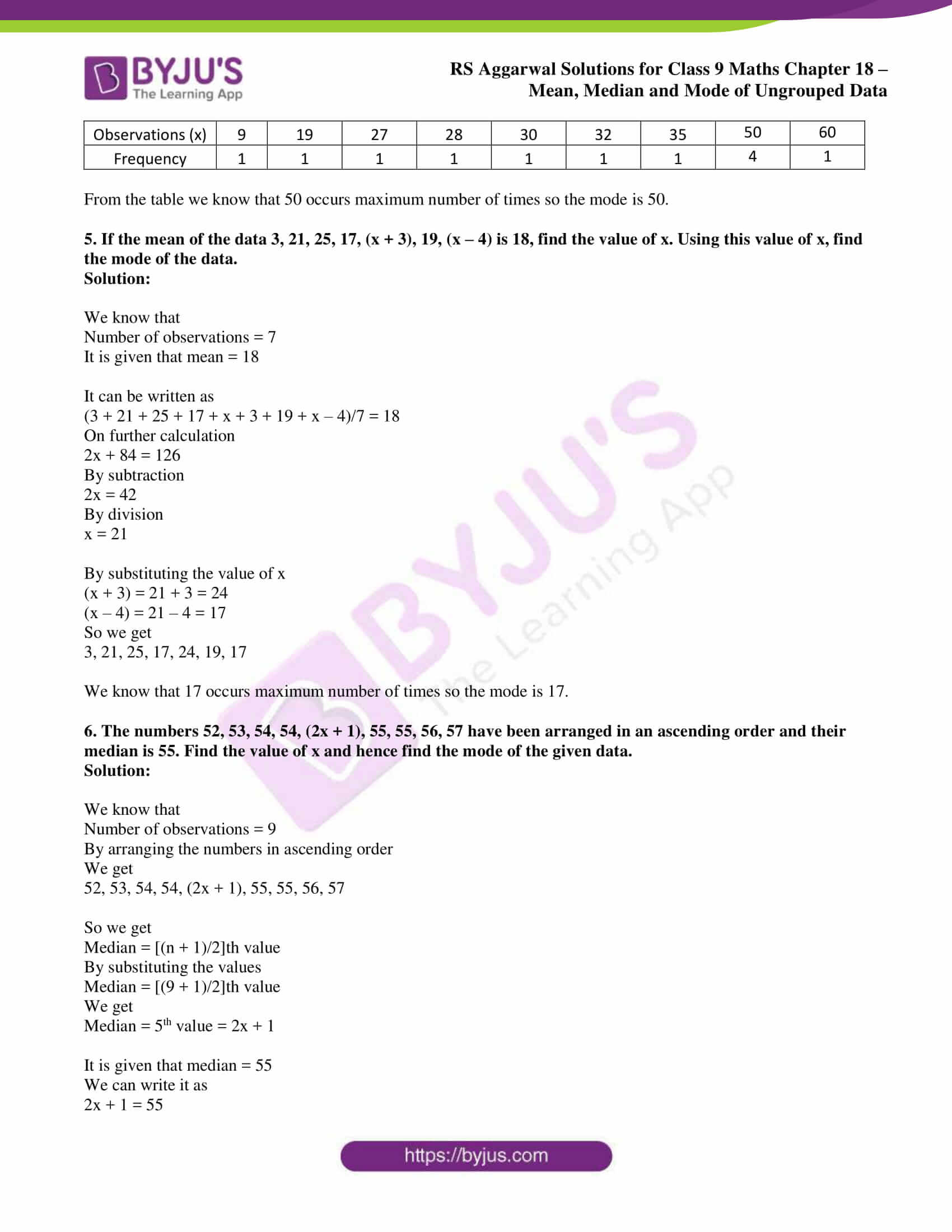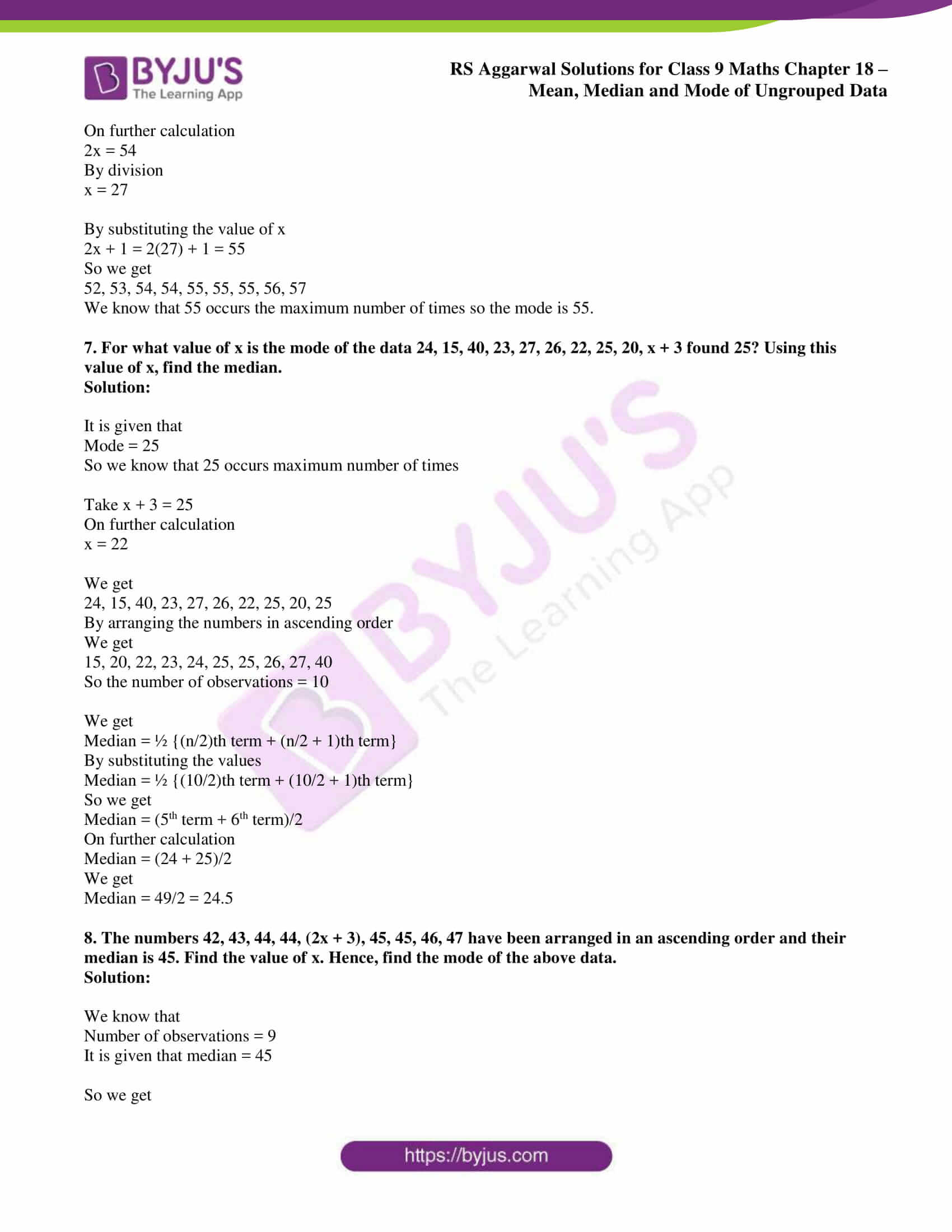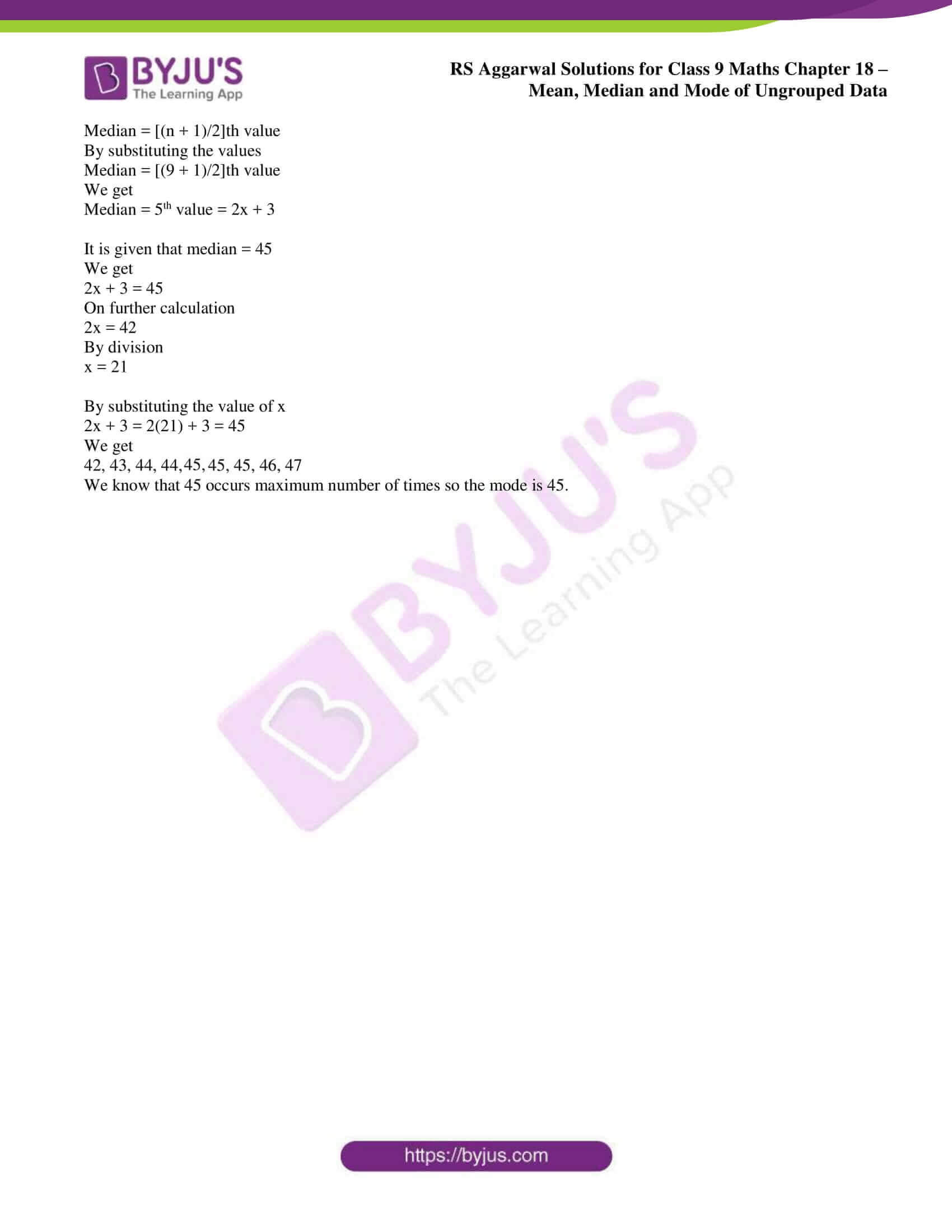### RS Aggarwal Solutions Class 9 Maths Chapter 18 – Exercise list

Exercise 18A Solutions 31 Questions

Exercise 18B Solutions 14 Questions

Exercise 18C Solutions 10 Questions

Exercise 18D Solutions 8 Questions

## Exercise 18(A)

1. Find the mean of:

(i) the first eight natural numbers

(ii) the first ten odd numbers

(iii) the first seven multiples of 5

(iv) all the factors of 20

(v) all prime numbers between 50 and 80.

Solution:

(i) We know that

First eight natural numbers = 1, 2, 3, 4, 5, 6, 7 and 8

So we get

Mean = sum of numbers/ total numbers

By substituting the values

Mean = (1 + 2 + 3 + 4 + 5 + 6 + 7 + 8)/8

On further calculation

Mean = 36/8

By division

Mean = 4.5

Therefore, the mean of the first eight natural numbers is 4.5.

(ii) We know that

First ten odd numbers = 1, 3, 5, 7, 9, 11, 13, 15, 17 and 19

So we get

Mean = sum of numbers/ total numbers

By substituting the values

Mean = (1 + 3 + 5 + 7 + 9 + 11 + 13 + 15 + 17 + 19)/10

On further calculation

Mean = 100/10

By division

Mean = 10

Therefore, the mean of first ten odd numbers is 10.

(iii) We know that

First seven multiples of five = 5, 10, 15, 20, 25, 30 and 35

So we get

Mean = sum of numbers/ total numbers

By substituting the values

Mean = (5 + 10 + 15 + 20 + 25 + 30 + 35)/7

On further calculation

Mean = 140/7

By division

Mean = 20

Therefore, the mean of first seven multiples of five is 20.

(iv) We know that

All the factors of 20 = 1, 2, 4, 5, 10 and 20

So we get

Mean = sum of numbers/ total numbers

By substituting the values

Mean = (1 + 2 + 4 + 5 + 10 + 20)/6

On further calculation

Mean = 42/6

By division

Mean = 7

Therefore, the mean of all the factors of 20 is 7.

(v) We know that

All prime numbers between 50 and 80 = 53, 59, 61, 67, 71, 73 and 79

So we get

Mean = sum of numbers/ total numbers

By substituting the values

Mean = (53 + 59 + 61 + 67 + 71 + 73 + 79)/7

On further calculation

Mean = 463/7

So we get

Mean = 66 1/7

Therefore, the mean of all prime numbers between 50 and 80 is 66 1/7.

2. The number of children in 10 families of a locality are 2, 4, 3, 4, 2, 0, 3, 5, 1 and 6.

Find the mean number of children per family.

Solution:

It is given that number of children in 10 families of a locality are 2, 4, 3, 4, 2, 0, 3, 5, 1 and 6.

We know that

Mean = sum of numbers/ total numbers

By substituting the values

Mean = (2 + 4 + 3 + 4 + 2 + 0 + 3 + 5 + 1 + 6)/ 10

On further calculation

Mean = 30/10

By division

Mean = 3

Therefore, the mean number of children per family is 3.

3. The following are the numbers of books issued in a school library during a week:

105, 216, 322, 167, 273, 405 and 346.

Find the average number of books issued per day.

Solution:

It is given that the number of books issued are 105, 216, 322, 167, 273, 405 and 346.

We know that

Mean = sum of numbers/ total numbers

By substituting the values

Mean = (105 + 216 + 322 + 167 + 273 + 405 + 346)/ 7

On further calculation

Mean = 1834/7

By division

Mean = 262

Therefore, the average number of books issued per day is 262.

4. The daily minimum temperature recorded (in oF) at a place during six days of a week was as under:

 Monday 35.5 Tuesday 30.8 Wednesday 27.3 Thursday 32.1 Friday 23.8 Saturday 29.9

Find the mean temperature.

Solution:

We know that

Mean temperature = Sum of temperatures/ Number of days

By substituting the values

Mean temperature = (35.5 + 30.8 + 27.3 + 32.1 + 23.8 + 29.9)/6

On further calculation

Mean = 179.4/6

By division

Mean = 29.9 oF

Therefore, the mean temperature is 29.9 oF.

5. If the mean of five observations x, x + 2, x + 4, x + 6, x + 8 is 13, find the value of x and hence find the mean of the last three observations.

Solution:

We know that

Number of observations = 5

It is given that mean = 13

We can write it as

[x + (x + 2) + (x + 4) + (x + 6) + (x + 8)]/5 = 13

On further calculation

5x + 20 = 13 (5)

So we get

5x + 20 = 65

By subtraction

5x = 45

By division

x = 9

By substituting the value of x

We know that the last three observations are

9 + 4 = 13

9 + 6 = 15

9 + 8 = 17

We know that

Mean of last three observations = (13 + 15 + 17)/3

On further calculation

Mean of last three observations = 45/3

By division

Mean of last three observations = 15

Therefore, the mean of last three observations is 15.

6. The mean weight of 6 boys in a group is 48kg. The individual weights of five of them are 51kg, 45kg, 49kg, 46kg and 44kg. Find the weight of the sixth boy.

Solution:

It is given that

Mean weight of 6 boys = 48kg

So we get

Mean weight = sum of the weight of 6 boys/6 = 48

We know that

Sum of weight of six boys = 48 (6) = 288kg

So the sum of weight of 5 boys = (51 + 45 + 49 + 46 + 44) = 235kg

We get

Weight of sixth boy = sum of weight of six boys – sum of weight of 5 boys

By substituting the values

Weight of sixth boy = 288 – 235

By subtraction

Weight of sixth boy = 53kg

Therefore, the weight of sixth boy is 53 kg.

7. The mean of the marks scored by 50 students was found to be 39. Later on it was discovered that a score of 43 was misread as 23. Find the correct mean.

Solution:

It is given that

Mean of the marks of 50 students = 39

So we get

Sum of these marks = 39 (50) = 1950

We can write it as

Corrected sum of the marks = 1950 – wrong number + correct number

By substituting the values

Corrected sum of the marks = 1950 – 23 + 43

So we get

Corrected sum of the marks = 1970

So the correct mean = 1970/50

By division

Correct mean = 39.4

Therefore, the correct mean is 39.4.

8. The mean of 24 numbers is 35. If 3 is added to each number, what will be the new mean?

Solution:

Consider x1, x2, …….. x24 as the given numbers

So we get

Mean = (x1 + x2, …….. + x24)/24

It is given that mean = 35

(x1 + x2, …….. + x24)/24 = 35

By cross multiplication

x1 + x2, …….. + x24 = 840 ……. (1)

Take (x1 + 3), (x2 + 3), ……… (x24 + 3) as new numbers

So the mean of new numbers = [(x1 + 3) + (x2 + 3) + ……… + (x24 + 3)]/24

From equation (1) we get

[(x1 + 3) + (x2 + 3) + ……… + (x24 + 3)]/24 = (840 + 72)/24

On further calculation

[(x1 + 3) + (x2 + 3) + ……… + (x24 + 3)]/24 = 912/24

By division

Mean of new numbers = 38

Therefore, the mean of the new numbers is 38.

9. The mean of 20 numbers is 43. If 6 is subtracted from each of the numbers, what will be the new mean?

Solution:

Consider x1, x2, ……. x20 as the given numbers

So we get

Mean = (x1 + x2 + ……. + x20)/20

It is given that mean = 43

(x1 + x2 + ……. + x20)/20 = 43

By cross multiplication

x1 + x2 + ……. + x20 = 860 …… (1)

Take (x1 – 6), (x2 – 6) …… (x20 – 6) as the new numbers

So the mean of new numbers = [(x1 – 6) + (x2 – 6) + …… + (x20 – 6)]/20

From equation (1) we get

[(x1 – 6) + (x2 – 6) + …… + (x20 – 6)]/20 = (860 – 120)/20

On further calculation

[(x1 – 6) + (x2 – 6) + …… + (x20 – 6)]/20 = 740/20

By division

Mean of new numbers = 37

Therefore, the new mean of numbers is 37.

10. The mean of 15 numbers is 27. If each number is multiplied by 4, what will be the mean of the new numbers?

Solution:

Consider x1, x2, ……. x15 as the given numbers

So we get

Mean = (x1 + x2 + ……. + x15)/15

It is given that mean = 27

(x1 + x2 + ……. + x15)/15 = 27

By cross multiplication

x1 + x2 + ……. + x15 = 405 …… (1)

Take (x1 × 4), (x2 × 4), ……… (x15 × 4) as new numbers

So the mean of new numbers = [(x1 × 4) + (x2 × 4) + ……… + (x15 × 4)]/15

From equation (1) we get

[(x1 × 4) + (x2 × 4) + ……… + (x15 × 4)]/15 = (405 × 4)/ 15

On further calculation

Mean of new numbers = 1620/15 = 108

Therefore, the mean of new numbers is 108.

11. The mean of 12 numbers is 40. If each number is divided by 8, what will be the mean of the new numbers?

Solution:

Consider x1, x2, ……. x12 as the given numbers

So we get

Mean = [x1 + x2 + ……. + x12]/12

It is given that mean = 40

(x1 + x2 + ……. + x12)/12 = 40

By cross multiplication

x1 + x2 + ……. + x12 = 480 …… (1)

Take (x1 ÷ 8), (x2 ÷ 8), ……… (x12 ÷ 8) as new numbers

So the mean of new numbers = [(x1 ÷ 8) + (x2 ÷ 8) + ……… + (x12 ÷ 8)]/12

From equation (1) we get

[(x1 ÷ 8) + (x2 ÷ 8) + ……… + (x12 ÷ 8)]/12 = (480 ÷ 8)/12

On further calculation

Mean of new numbers = 60/12 = 5

Therefore, the mean of the new numbers is 5.

12. The mean of 20 numbers is 18. If 3 is added to each of the first ten numbers, find the mean of the new set of 20 numbers.

Solution:

Consider x1, x2, ……. x20 as the given numbers

So we get

Mean = (x1 + x2 + ……. + x20)/20

It is given that mean = 18

(x1 + x2, ……. + x20)/20 = 18

By cross multiplication

x1 + x2 + ……. + x20 = 360 …… (1)

Take (x1 + 3), (x2 + 3), ……… (x10 + 3) as first ten new numbers

So the mean of new set of 20 numbers = [(x1 + 3) + (x2 + 3) + ……… + (x10 + 3) + x11 + …….. + x20]/ 20

We get

Mean of new set of 20 numbers = [(x1 + x2 + ……. + x20) + 3 × 10]/ 20

From equation (1) we get

Mean of new set of 20 numbers = (360 + 30)/ 20 = 19.5

Therefore, the mean of new set of 20 numbers is 19.5.

13. The mean of six numbers is 23. If one of the numbers is excluded, the mean of the remaining numbers is 20. Find the excluded number.

Solution:

It is given that

Mean of six numbers = 23

So we get the sum of six numbers = 23 (6) = 138

It is given that

Mean of five numbers = 20

So we get the sum of five numbers = 20 (5) = 100

We know that

Excluded number = sum of six numbers – sum of five numbers

By substituting the values

Excluded number = 138 – 100 = 38

Therefore, the excluded number is 38.

14. The average height of 30 boys was calculated to be 150cm. It was detected later that one value of 165cm was wrongly copied as 135cm for the computation of the mean. Find the correct mean.

Solution:

It is given that

Mean height of 30 boys = 150cm

So the total height = 150 (30) = 4500cm

We know that

Correct sum = 4500 – incorrect value + correct value

By substituting the values

Correct sum = 4500 – 135 + 165 = 4350

So we get

Correct mean = Correct sum/30

By substituting the values

Correct mean = 4530/30 = 151 cm

Therefore, the correct mean is 151 cm.

15. The mean weight of a class of 34 students is 46.5kg. If the weight of the teacher is included, the mean rises by 500g. Find the weight of the teacher.

Solution:

It is given that

Mean weight of 34 students = 46.5kg

So the total weight = 34 (46.5) = 1581kg

We know that

Mean weight of 34 students and teacher = 46.5 + 0.5 = 47kg

So the total weight of 34 students and teacher = 47 (35) = 1645kg

The weight of teacher = Weight of 34 students and teacher – total weight

By substituting the values

Weight of the teacher = 1645 – 1581 = 64kg

Therefore, the weight of the teacher is 64kg.

16. The mean weight of a class of 36 students is 41kg. If one of the students leaves the class then the mean is decreased by 200g. Find the weight of the student who left.

Solution:

It is given that

Mean weight of 36 students = 41kg

So the total weight = 41 (36) = 1476kg

It is given that if one of the students leaves the class then the mean is decreased by 200g

So the new mean = 41 – 0.2 = 40.8kg

We get the total weight of 35 students = 40.8 (35) = 1428kg

So the weight of student who left = total weight of 36 students – weight of 35 students

By substituting the values

Weight of the student who left the class = 1476 – 1428 = 48kg

Therefore, the weight of the student who left is 48kg.

17. The average weight of a class of 39 students is 40kg. When a new student is admitted to the class, the average decreases by 200g. Find the weight of the new student.

Solution:

It is given that

Mean weight of 39 students = 40kg

So the total weight = 40 (39) = 1560kg

It is given that when a new student is admitted to the class, the average decreases by 200g

So the new mean = 40 – 0.2 = 39.8kg

We know that

Total weight of 40 students = 39.8 (40) = 1592kg

So the weight of new student = total weight of 40 students – total weight of 39 students

By substituting the values

Weight of new student = 1592 – 1560 = 32kg

Therefore, the weight of the new student is 32kg.

18. The average weight of 10 oarsmen in a boat is increased by 1.5kg when one of the crew who weighs 58kg is replaced by a new man. Find the weight of the new man.

Solution:

It is given that

Weight of 10 oarsmen is increased by 1.5kg

So the total weight increased = 1.5 (10) = 15kg

It is given that one of the crew who weights 58kg is replaced by a new man

So we get the weight of new man = weight of man replaced + total weight increased

By substituting the values

Weight of the new man = 58 + 15 = 73kg

Therefore, the weight of a new man is 73kg.

19. The mean of 8 numbers is 35. If a number is excluded then the mean is reduced by 3. Find the excluded number.

Solution:

It is given that

Mean of 8 numbers = 35

So the total sum = 35 (8) = 280

It is given that if a number is excluded then the mean is reduced by 3

So the new mean = 35 – 3 = 32

We know that total sum of 7 numbers = 32 (7) = 224

So we get

Excluded number = sum of 8 numbers – sum of 7 numbers

By substituting the values

Excluded number = 280 – 224 = 56

Therefore, the excluded number is 56.

20. The mean of 150 items was found to be 60. Later on, it was discovered that the values of two items were misread are 52 and 8 instead of 152 and 88 respectively. Find the correct mean.

Solution:

It is given that

Mean of 150 items = 60

So the total sum = 150 (60) = 9000

We know that

Correct sum of items = sum of 150 items – sum of wrong items + sum of right items

By substituting the values

Correct sum of items = 90000 – (52 + 8) + (152 + 88)

On further calculation

Correct sum of items = 9000 – 60 + 240

So we get

Correct sum of items = 9180

So the correct mean = correct sum of items/ total number of items

By substituting the values

Correct mean = 9180/150 = 61.2

Therefore, the correct mean is 61.2.

21. The mean of 31 results is 60. If the mean of the first 16 results is 58 and that of the last 16 results is 62, find the 16th result.

Solution:

It is given that

Mean of 31 results = 60

So the total sum = 31 (60) = 1860

We know that

Mean of first 16 results = 58

So the total sum = 16 (58) = 928

Mean of last 16 results = 62

So the total sum = 16 (62) = 992

We get the 16th result = total sum of first 16 results + total sum of last 16 results – total sum of 31 results

By substituting the values

16th result = 928 + 992 – 1860

On further calculation

16th result = 1920 – 1860 = 60

Therefore, the 16th result is 60.

22. The mean of 11 numbers is 42. If the mean of the first 6 numbers is 37 and that of the last 6 numbers is 46, find the 6th number.

Solution:

It is given that

Mean of 11 numbers = 42

So the total sum = 42 (11) = 462

Mean of first 6 numbers = 37

So the total sum = 37 (6) = 222

Mean of last 6 numbers = 46

So the total sum = 6 (46) = 276

We know that

6th number = total sum of last 6 numbers + total sum of first 6 numbers – total sum of 11 numbers

By substituting the values

6th number = 276 + 222 – 462

So we get

6th number = 498 – 462 = 36

Therefore, the 6th number is 36.

23. The mean weight of 25 students of a class is 52kg. If the mean weight of the first 13 students of the class is 48kg and that of the last 13 students is 55kg, find the weight of the 13th student.

Solution:

It is given that

Mean weight of 25 students = 52kg

So the total weight = 25 (52) = 1300kg

Mean weight of first 13 students = 48kg

So the total weight = 13 (48) = 624kg

Mean weight of last 13 students = 55kg

So the total weight = 13 (55) = 715kg

We know that

Weight of 13th student = total weight of the first 13 students + total weight of last 13 students – total weight of 25 students

By substituting the values

Weight of 13th student = 624 + 715 – 1300

On further calculation

Weight of 13th student = 39kg

Therefore, the weight of the 13th student is 39kg.

24. The mean score of 25 observations is 80 and the mean score of another 55 observations is 60. Determine the mean score of the whole set of observations.

Solution:

It is given that

Mean score of 25 observations = 80

So the total score = 25 (80) = 2000

Mean score of 55 observations = 60

So the total score = 55 (60) = 3300

We know that

Total number of observations = 25 + 55 = 80

So the total score = 2000 + 3300 = 5300

Mean score = total score/total number of observations

By substituting the values

Mean score = 5300/80

So we get

Mean score = 66.25

Therefore, the mean score of the whole set of observations is 66.25.

25. Arun scored 36 marks in English, 44 marks in Hindi, 74 marks in mathematics and x marks in science. If he has secured an average of 50 marks, find the value of x.

Solution:

It is given that

Average marks in 4 subjects = 50

So the total marks = 50 (4) = 200

We know that

36 + 44 + 75 + x = 200

On further calculation

155 + x = 200

So we get

x = 200 – 155

By subtraction

x = 45

Therefore, the value of x is 45.

26. A ship sails out to an island at the rate of 15 km/hr and sails back to the starting point at 10 km/hr. Find the average sailing speed for the whole journey.

Solution:

Consider x km as the distance of mark from the starting point

So the time taken by the ship to reach the mark = x/15 hours

We know that

The time taken by the ship to reach the starting point from the mark = x/10 hours

So we get

Total time taken = x/15 + x/10

By taking LCM

Total time taken = x/6 hours

We know that total distance covered = x + x = 2x km

So the average sailing speed for the whole journey = 2x ÷ x/6

It can be written as

Average sailing speed for the whole journey = (2x × 6)/ x

So we get

Average sailing speed for the whole journey = 12 km/hr

Therefore, the average sailing speed for the whole journey is 12 km/hr.

27. There are 50 students in a class, of which 40 are boys. The average weight of the class is 44kg and that of the girls is 40kg. Find the average weight of the boys.

Solution:

It is given that

Total number of students = 50

So total number of girls = 50 – 40 = 10

Average weight of the class = 44kg

So the total weight = 44 (50) = 2200kg

Average weight of 10 girls = 40kg

So total weight = 40 (10) = 400kg

We know that total weight of 40 boys = total weight of 50 students – total weight of 10 girls

By substituting the values

Total weight of 40 boys = 2200 – 400 = 1800kg

So we get

Average weight of boys = total weight of 40 boys/ number of boys

By substituting the values

Average weight of boys = 1800/40 = 45kg

Therefore, the average weight of boys = 45kg.

28. The aggregate monthly expenditure of a family was ₹ 18720 during the first 3 months, ₹ 20340 during the next 4 months and ₹ 21708 during the last 5 months of a year. If the total savings during the year be ₹ 35340 find the average monthly income of the family.

Solution:

We know that

Total earnings of the year = ₹ (3 × 18720 + 4 × 20340 + 5 × 21708 + 35340)

On further calculation

Total earnings of the year = ₹ (56160 + 81360 + 108540 + 35340)

So we get

Total earnings of the year = ₹ 281400

We know that

Average monthly income of the family = Total earning of the year/ Number of months

By substituting the values

Average monthly income of the family = 281400/ 12

So we get

Average monthly income of the family = ₹ 23450

Therefore, the average monthly income of the family is ₹ 23450.

29. The average weekly payment to 75 workers in a factory is ₹ 5680. The mean weekly payment to 25 of them is ₹ 5400 and that of 30 others is ₹ 5700. Find the mean weekly payment of the remaining workers.

Solution:

It is given that

Average weekly payment of 75 workers = ₹ 5680

So the total payment = ₹ (75 × 5680) = ₹ 426000

Mean weekly payment of 25 workers = ₹ 5400

So the total payment = ₹ (25 × 5400) = ₹ 135000

Mean weekly payment of 30 workers = ₹ 5700

So the total payment = ₹ (30 × 5700) = ₹ 171000

We know that number of remaining workers = 75 – 25 – 30 = 20

So the total weekly payment of remaining 20 workers = Total weekly payment of 75 workers – total weekly payment of 25 workers – total weekly payment of 30 workers

By substituting the values

Total weekly payment of remaining 2 workers = 426000 – 135000 – 171000 = ₹ 120000

So the mean weekly payment of remaining 20 workers = 120000/20 = ₹ 6000

Therefore, the mean weekly payment of the remaining workers is ₹ 6000.

30. The mean marks (out of 100) of boys and girls in an examination are 70 and 73 respectively. If the mean marks of all the students in that examination is 71, find the ratio of the number of boys to the number of girls.

Solution:

Consider x: 1 as the ratio of number of boys to the number of girls

So we get

Sum of marks of boys = 70x

Sum of marks of girls = 73 (1) = 73

We know that

Sum of marks of boys and girls = 71 (x + 1)

It can be written as

70x + 73 = 71 (x + 1)

On further calculation

70x + 73 = 71x + 71

So we get

x = 2

Therefore, the ratio of the number of boys to the number of girls is 2: 1.

31. The average monthly salary of 20 workers in an office is ₹ 45900. If the manager’s salary is added, the average salary becomes ₹ 49200 per month. What’s manager’s monthly salary?

Solution:

It is given that

Mean monthly salary of 20 workers = ₹ 45900

So the total monthly salary = ₹ (20 × 49500) = ₹ 918000

Mean monthly salary of 20 workers and manager = ₹ 49200

So the total monthly salary = ₹ (21 × 49200) = ₹ 1033200

We know that

Manager’s monthly salary = Total monthly salary of 20 workers and manager – Total monthly salary of 20 workers

By substituting the values

Manager’s monthly salary = 1033200 – 918000 = ₹ 115200

Therefore, the manager’s monthly salary is ₹ 115200.

## Exercise 18(B)

1. Obtain the mean of the following distribution:

 Variable (xi) 4 6 8 10 12 Frequency (fi) 4 8 14 11 3

Solution:

 Variable (xi) Frequency (fi) fi xi 4 4 16 6 8 48 8 14 112 10 11 110 12 3 36 Σ fi = 40 Σ fi xi = 322

We know that

Mean = Σ fi xi/ Σ fi

By substituting the values

Mean = 322/40 = 8.05

2. The following table shows the weights of 12 workers in a factory:

 Weight (in kg) 60 63 66 69 72 No. of workers 4 3 2 2 1

Find the mean weight of the workers.

Solution:

 Weight (in kg) (xi) No. of workers (fi) fi xi 60 4 240 63 3 189 66 2 132 69 2 138 72 1 72 Σ fi = 12 Σ fi xi =771

We know that

Mean weight of the workers = Σ fi xi/ Σ fi

By substituting the values

Mean weight of the workers = 771/12 = 64.250kg

Therefore, the mean weight of the workers is 64.250kg.

3. The measurements (in mm) of the diameters of the heads of 50 screws are given below:

 Diameter (in mm) (xi) 34 37 40 43 46 Number of screws (fi) 5 10 17 12 6

Calculate the mean diameter of the heads of the screws.

Solution:

 Diameter (in mm) (xi) Number of screws (fi) fi xi 34 5 170 37 10 370 40 17 680 43 12 516 46 6 276 Σ fi = 50 Σ fi xi = 2012

We know that

Mean diameter of the heads of the screws = Σ fi xi/ Σ fi

By substituting the values

Mean diameter of the heads of the screws = 2012/50 = 40.24mm

Therefore, the mean diameter of the heads of the screws is 40.24mm.

4. The following data give the number of boys of a particular age in a class of 40 students.

 Age (in years) 15 16 17 18 19 20 Frequency (fi) 3 8 9 11 6 3

Calculate the mean age of the students.

Solution:

 Age (in years) (xi) Frequency (fi) fi xi 15 3 45 16 8 128 17 9 153 18 11 198 19 6 114 20 3 60 Σ fi = 40 Σ fi xi = 698

We know that

Mean age of the students = Σ fi xi/ Σ fi

By substituting the values

Mean age of the students = 698/40 = 17.45 years

Therefore, the mean age of the students is 17.45 years.

5. Find the mean of the following frequency distribution:

 Variables (xi) 10 30 50 70 89 Frequency (fi) 7 8 10 15 10

Solution:

 Variables (xi) Frequency (fi) fi xi 10 7 70 30 8 240 50 10 500 70 15 1050 89 10 890 Σ fi = 50 Σ fi xi = 2750

We know that

Mean = Σ fi xi/ Σ fi

By substituting the values

Mean = 2750/50 = 55

6. Find the mean of daily wages of 40 workers in a factory as per data given below:

 Daily wages (in ₹ ) (xi) 250 300 350 400 450 Number of workers (fi) 8 11 6 10 5

Solution:

 Daily wages (in ₹ ) (xi) Number of workers (fi) fi xi 250 8 2000 300 11 3300 350 6 2100 400 10 4000 450 5 2250 Σ fi = 40 Σ fi xi = 13650

We know that

Mean of daily wages of 40 workers in a factory = Σ fi xi/ Σ fi

By substituting the values

Mean of daily wages of 40 workers in a factory = 13650/40 = ₹ 341.25

Therefore, the mean of daily wages of 40 workers in a factory is ₹ 341.25.

7. If the mean of the following data is 20.2, find the value of p.

 Variable (xi) 10 15 20 25 30 Frequency (fi) 6 8 p 10 6

Solution:

 Variable (xi) Frequency (fi) fi xi 10 6 60 15 8 120 20 p 20p 25 10 250 30 6 180 Σ fi = 30 + p Σ fi xi = 610 + 20p

It is given that

Mean = 20.2

We know that

Mean = Σ fi xi/ Σ fi

So we get

(610 + 20p)/ (30 + p) = 20.2

On further calculation

610 + 20p = 606 + 20.2p

So we get

0.2p = 4

By division

p = 20

Therefore, the value of p is 20.

8. If the mean of the following data is 8, find the value of p.

 x 3 5 7 9 11 13 f 6 8 15 p 8 4

Solution:

 x f fi xi 3 6 18 5 8 40 7 15 105 9 p 9p 11 8 88 13 4 52 Σ fi = 41 + p Σ fi xi = 303 + 9p

It is given that

Mean = 8

We know that

Mean = Σ fi xi/ Σ fi

So we get

(303 + 9p)/ (41 + p) = 8

On further calculation

303 + 9p = 8 (41 + p)

So we get

303 + 9p = 328 + 8p

It can be written as

9p – 8p = 328 – 303

We get

p = 25

Therefore, the value of p is 25.

9. Find the missing frequency p for the following frequency distribution whose mean is 28.25.

 x 15 20 25 30 35 40 f 8 7 p 14 15 6

Solution:

 x f fi xi 15 8 120 20 7 140 25 p 25p 30 14 420 35 15 525 40 6 240 Σ fi = 50 + p Σ fi xi = 1445 + 25p

It is given that

Mean = 28.25

We know that

Mean = Σ fi xi/ Σ fi

So we get

(1445 + 25p)/ (50 + p) = 28.25

On further calculation

1445 + 25p = 1412.50 + 28.25p

So we get

-28.25p + 25p = -1445 + 1412.50

It can be written as

-3.25p = -32.5

By division

p = 10

Therefore, the value of p is 10.

10. Find the value of p for the following frequency distribution whose mean is 16.6.

 x 8 12 15 p 20 25 30 f 12 16 20 24 16 8 4

Solution:

 x f fi xi 8 12 96 12 16 192 15 20 300 p 24 24p 20 16 320 25 8 200 30 4 120 Σ fi = 100 Σ fi xi = 1228 + 24p

It is given that

Mean = 16.6

We know that

Mean = Σ fi xi/ Σ fi

So we get

(1228 + 24p)/ 100 = 16.6

On further calculation

1228 + 24p = 1660

So we get

24p = 1660 – 1228

By subtraction

24p = 432

We get

p = 18

Therefore, the value of p is 18.

11. Find the missing frequencies in the following frequency distribution whose mean is 34.

 x 10 20 30 40 50 6 Total f 4 f1 8 f2 3 4 35

Solution:

 x f fi xi 10 4 40 20 f1 20f1 30 8 240 40 f2 40f2 50 3 150 6 4 240 Total 35 = Σ fi =19 + f1 + f2 Σ fi xi = 670 + 20f1 + 40f2

We know that

Σ fi =19 + f1 + f2

It can be written as

35 = 19 + f1 + f2

So we get

f1 + f2 = 16 …… (1)

It is given that

Mean = 34

We know that

Mean = Σ fi xi/ Σ fi

So we get

(670 + 20f1 + 40f2)/ 35 = 34

On further calculation

(335 + 10f1 + 20f2)/ 35 = 17

So we get

335 + 10f1 + 20f2 = 595

It can be written as

10f1 + 20f2 = 260

Dividing the equation by 10

f1 + 2f2 = 26 …….. (2)

By subtracting equation (1) from (2)

f2 = 10

Substituting in equation (1)

f1 + 10 = 16

So we get

f1 = 6

Therefore, the missing frequencies are 6 and 10.

12. Find the missing frequencies in the following frequency distribution whose mean is 50.

 x 10 30 50 70 90 Total f 17 f1 32 f2 19 120

Solution:

 x f fi xi 10 17 170 30 f1 30f1 50 32 1600 70 f2 70f2 90 19 1710 Total 120 = Σ fi =68 + f1 + f2 Σ fi xi = 3480 + 30f1 + 70f2

We know that

Σ fi =68 + f1 + f2

It can be written as

120 = 68 + f1 + f2

So we get

f1 + f2 = 52

f2 = 52 – f1 ……. (1)

It is given that

Mean = 50

We know that

Mean = Σ fi xi/ Σ fi

So we get

(3480 + 30f1 + 70f2)/ 120 = 50

Substituting equation (1)

3480 + 30f1 + 70(52 – f1) / 120 = 50

On further calculation

(3480 + 30f1 + 3640 – 70f1)/ 120 = 50

So we get

(7120 – 40f1)/ 120 = 50

It can be written as

6000 = 7120 – 40f1

By subtraction

40f1 = 1120

By division

f1 = 28

By substituting it in equation (1)

f2 = 52 – 28 = 24

Therefore, the missing frequencies are 28 and 24.

13. Find the value of p, when the mean of the following distribution is 20.

 x 15 17 19 20 + p 23 f 2 3 4 5p 6

Solution:

 x f fi xi 15 2 30 17 3 51 19 4 76 20 + p 5p 100p + 5p2 23 6 138 Σ fi = 15 + 5p Σ fi xi = 5p2 + 100p + 295

It is given that

Mean = 20

We know that

Mean = Σ fi xi/ Σ fi

So we get

(5p2 + 100p + 295)/ (15 + 5p) = 20

On further calculation

5p2 + 100p + 295 = 300 + 100p

So we get

5p2 = 5

By division

p2 = 1

By taking square root

p = 1

Therefore, the value of p is 1.

14. The mean of the following distribution is 50.

 x 10 30 50 70 90 f 17 5a + 3 32 7a – 11 19

Find the value of a and hence the frequencies of 30 and 70.

Solution:

 x f fi xi 10 17 170 30 5a + 3 150a + 90 50 32 1600 70 7a – 11 490a – 770 90 19 171 Σ fi = 60 + 12a Σ fi xi = 2800 + 640a

It is given that

Mean = 50

We know that

Mean = Σ fi xi/ Σ fi

So we get

(2800 + 640a)/ (60 + 12a) = 50

On further calculation

2800 + 640a = 3000 + 600a

So we get

40a = 200

By division

a = 5

We know that

Frequency of 30 = 5a + 3

By substituting the values

Frequency of 30 = 5 (5) + 3

So we get

Frequency of 30 = 28

We know that

Frequency of 70 = 7a – 11

By substituting the values

Frequency of 70 = 7 (5) – 11

So we get

Frequency of 70 = 24

Therefore, the value of a is 5 and the frequencies of 30 and 70 is 28 and 24.

## Exercise 18(C)

1. Find the median of:

(i) 2, 10, 9, 9, 5, 2, 3, 7, 11

(ii) 15, 6, 16, 8, 22, 21, 9, 18, 25

(iii) 20, 13, 18, 25, 6, 15, 21, 9, 16, 8, 22

(iv) 7, 4, 2, 5, 1, 4, 0, 10, 3, 8, 5, 9, 2

Solution:

(i) By arranging the numbers in ascending order

We get

2, 2, 3, 5, 7, 9, 9, 10, 11

We know that n = 9 is odd

So we get

Median = ½ (n + 1) th term

By substituting the values

Median = ½ (9 + 1) th term

It can be written as

Median = value of the 5th term = 7

We get

Median = 7

(ii) By arranging the numbers in ascending order

We get

6, 8, 9, 15, 16, 18, 21, 22, 25

We know that n = 9 is odd

So we get

Median = ½ (n + 1) th term

By substituting the values

Median = ½ (9 + 1) th term

It can be written as

Median = value of the 5th term = 16

We get

Median = 16

(iii) By arranging the numbers in ascending order

We get

6, 8, 9, 13, 15, 16, 18, 20, 21, 22, 25

We know that n = 11 is odd

So we get

Median = ½ (n + 1) th term

By substituting the values

Median = ½ (11 + 1) th term

It can be written as

Median = value of the 6th term = 16

We get

Median = 16

(iv) By arranging the numbers in ascending order

We get

0, 1, 2, 2, 3, 4, 4, 5, 5, 7, 8, 9, 10

We know that n = 13 is odd

So we get

Median = ½ (n + 1) th term

By substituting the values

Median = ½ (13 + 1) th term

It can be written as

Median = value of the 7th term = 4

We get

Median = 4

2. Find the median of:

(i) 17, 19, 32, 10, 22, 21, 9, 35

(ii) 72, 63, 29, 51, 35, 60, 55, 91, 85, 82

(iii) 10, 75, 3, 15, 9, 47, 12, 48, 4, 81, 17, 27

Solution:

(i) By arranging the numbers in ascending order

We get

9, 10, 17, 19, 21, 22, 32, 35

We know that n = 8 which is even

So we get

Median = ½ {(n/2)th term + (n/2 + 1)th term}

It can be written as

Median = ½ {4th term + 5th term)

By substituting the values

Median = ½ (19 + 21)

On further calculation

Median = ½ (40)

We get

Median = 20

(ii) By arranging the numbers in ascending order

We get

29, 35, 51, 55, 6, 63, 72, 82, 85, 91

We know that n = 10 which is even

So we get

Median = ½ {(n/2)th term + (n/2 + 1)th term}

It can be written as

Median = ½ {5th term + 6th term)

By substituting the values

Median = ½ (60 + 63)

On further calculation

Median = ½ (123)

We get

Median = 61.5

(iii) By arranging the numbers in ascending order

We get

3, 4, 9, 10, 12, 15, 17, 27, 47, 48, 75, 81

We know that n = 12 which is even

So we get

Median = ½ {(n/2)th term + (n/2 + 1)th term}

It can be written as

Median = ½ {6th term + 7th term)

By substituting the values

Median = ½ (15 + 17)

On further calculation

Median = ½ (32)

We get

Median = 16

3. The marks of 15 students in an examination are

25, 19, 17, 24, 23, 29, 31, 4, 19, 20, 22, 26, 17, 25, 21.

Find the median score.

Solution:

By arranging the numbers in ascending order

We get

17, 17, 19, 19, 20, 21, 22, 23, 24, 25, 26, 29, 31, 35, 40

We know that n = 15 which is odd

So we get

Median = ½ (n + 1) th term

By substituting the values

Median = ½ (15 + 1) th term

It can be written as

Median = value of the 8th term = 23

Therefore, the median score is 23.

4. The heights (in cm) of 9 students of a class are

148, 144, 152, 155, 160, 147, 150, 149, 145.

Find the median height.

Solution:

By arranging the numbers in ascending order

We get

144, 145, 147, 148, 149, 150, 152, 155, 160

We know that n = 9 which is odd

So we get

Median = [(n + 1)/2]th value

By substituting the values

Median = [(9 + 1)/2]th value

We get

Median = 5th value = 149cm

Therefore, the median height is 149cm.

5. The weights (in kg) of 8 children are

13.4, 10.6, 12.7, 17.2, 14.3, 15, 16.5, 9.8.

Find the median weight.

Solution:

By arranging the numbers in ascending order

We get

9.8, 1.6, 12.7, 13.4, 14.3, 15, 16.5, 17.2

We know that n = 8 which is even

So we get

Median = ½ {(n/2)th term + (n/2 + 1)th term}

It can be written as

Median = ½ {4th term + 5th term)

By substituting the values

Median = ½ (13.4 + 14.3)

On further calculation

Median = ½ (27.7)

We get

Median = 13.85kg

Therefore, the median weight is 13.85kg.

6. The ages (in years) of 10 teachers in a school are

32, 44, 53, 47, 37, 54, 34, 36, 40, 50.

Find the median age.

Solution:

By arranging the numbers in ascending order

We get

32, 34, 36, 37, 4, 44, 47, 50, 53, 54

We know that n = 10 which is even

So we get

Median = ½ {(n/2)th term + (n/2 + 1)th term}

It can be written as

Median = ½ {5th term + 6th term)

By substituting the values

Median = ½ (40 + 44)

On further calculation

Median = ½ (84)

We get

Median = 42

Therefore, the median age is 42 years.

7. If 10, 13, 15, 18, x + 1, x + 3, 30, 32, 35, 41 are ten observations in an ascending order with median 24, find the value of x.

Solution:

By arranging the numbers in ascending order

We get

10, 13, 15, 18, x + 1, x + 3, 30, 32, 35, 41

We know that n = 10 which is even

So we get

Median = ½ {(n/2)th term + (n/2 + 1)th term}

It can be written as

Median = ½ {5th term + 6th term)

By substituting the values

Median = ½ (x + 1 + x + 3)

On further calculation

Median = ½ (2x + 4)

We get

Median = x + 2

It is given that median = 24

So we get

x + 2 = 24

On further calculation

x = 24 – 2 = 22

Therefore, the value of x is 22.

8. The following observations are arranged in ascending order:

26, 29, 42, 53, x, x + 2, 70, 75, 82, 93.

If the median is 65, find the value of x.

Solution:

We know that n = 10 which is even

It is given that median = 65

We can write it as

½ {(n/2)th term + (n/2 + 1)th term} = 65

By substituting the values

½ {(10/2)th term + (10/2 + 1)th term} = 65

So we get

½ {5th value + 6th value} = 65

It can be written as

(x + x + 2)/2 = 65

On further calculation

2x + 2 = 130

By subtraction

2x = 128

By division

x = 64

Therefore, the value of x is 64.

9. The numbers 50, 42, 35, (2x + 10), (2x – 8), 12, 11, 8 have been written in a descending order. If their median is 25, find the value of x.

Solution:

We know that n = 8 which is even

It is given that median = 25

We can write it as

½ {(n/2)th term + (n/2 + 1)th term} = 25

By substituting the values

½ {(8/2)th term + (8/2 + 1)th term} = 25

So we get

½ {4th value + 5th value} = 25

It can be written as

(2x + 10 + 2x – 8)/2 = 25

On further calculation

4x + 2 = 50

By subtraction

4x = 48

By division

x = 12

Therefore, the value of x is 12.

10. Find the median of the data

46, 41, 77, 58, 35, 64, 87, 92, 33, 55, 90.

In the above data, if 41 and 55 are replaced by 61 and 75 respectively, what will be the new median?

Solution:

We know that n = 11 which is odd

By arranging the numbers in ascending order

We get

33, 35, 41, 46, 55, 58, 64, 77, 87, 90, 92

So we get

Median = [(n + 1)/2]th value

By substituting the values

Median = [(11 + 1)/2]th value

We get

Median = 6th value = 58

By replacing 41 and 55 by 61 and 75

By arranging the numbers in ascending order

We get

33, 35, 46, 58, 61, 64, 75, 77, 87, 90, 92

So we get

Median = [(n + 1)/2]th value

By substituting the values

Median = [(11 + 1)/2]th value

We get

Median = 6th value = 64

Therefore, the new median is 64.

## Exercise 18(D)

1. Find the mode of the following items.

0, 6, 5, 1, 6, 4, 3, 0, 2, 6, 5, 6

Solution:

By arranging the numbers in ascending order

We get

0, 0, 1, 2, 3, 4, 5, 5, 6, 6, 6, 6

 Observations (x) 0 1 2 3 4 5 6 Frequency 2 1 1 1 1 2 4

From the table we know that 6 occurs maximum number of times so the mode is 6.

2. Determine the mode of the following values of a variable.

23, 15, 25, 40, 27, 25, 22, 25, 20

Solution:

By arranging the numbers in ascending order

We get

15, 20, 22, 23, 25, 25, 25, 27, 40

 Observations (x) 15 20 22 23 25 27 40 Frequency 1 1 1 1 3 1 1

From the table we know that 25 occurs maximum number of times so the mode is 25.

3. Calculate the mode of the following sizes of shoes sold by a shop on a particular day.

5, 9, 8, 6, 9, 4, 3, 9, 1, 6, 3, 9, 7, 1, 2, 5, 9

Solution:

By arranging the numbers in ascending order

We get

1, 1, 2, 3, 3, 4, 5, 5, 6, 6, 7, 8, 9, 9, 9, 9, 9

 Observations (x) 1 2 3 4 5 6 7 8 9 Frequency 2 1 2 1 2 2 1 1 5

From the table we know that 9 occurs maximum number of times so the mode is 9.

4. A cricket player scored the following runs in 12 one-day matches:

50, 30, 9, 32, 60, 50, 28, 50, 19, 50, 27, 35.

Find his modal score.

Solution:

By arranging the numbers in ascending order

We get

9, 19, 27, 28, 30, 32, 35, 50, 50, 50, 50, 60

 Observations (x) 9 19 27 28 30 32 35 50 60 Frequency 1 1 1 1 1 1 1 4 1

From the table we know that 50 occurs maximum number of times so the mode is 50.

5. If the mean of the data 3, 21, 25, 17, (x + 3), 19, (x – 4) is 18, find the value of x. Using this value of x, find the mode of the data.

Solution:

We know that

Number of observations = 7

It is given that mean = 18

It can be written as

(3 + 21 + 25 + 17 + x + 3 + 19 + x – 4)/7 = 18

On further calculation

2x + 84 = 126

By subtraction

2x = 42

By division

x = 21

By substituting the value of x

(x + 3) = 21 + 3 = 24

(x – 4) = 21 – 4 = 17

So we get

3, 21, 25, 17, 24, 19, 17

We know that 17 occurs maximum number of times so the mode is 17.

6. The numbers 52, 53, 54, 54, (2x + 1), 55, 55, 56, 57 have been arranged in an ascending order and their median is 55. Find the value of x and hence find the mode of the given data.

Solution:

We know that

Number of observations = 9

By arranging the numbers in ascending order

We get

52, 53, 54, 54, (2x + 1), 55, 55, 56, 57

So we get

Median = [(n + 1)/2]th value

By substituting the values

Median = [(9 + 1)/2]th value

We get

Median = 5th value = 2x + 1

It is given that median = 55

We can write it as

2x + 1 = 55

On further calculation

2x = 54

By division

x = 27

By substituting the value of x

2x + 1 = 2(27) + 1 = 55

So we get

52, 53, 54, 54, 55, 55, 55, 56, 57

We know that 55 occurs the maximum number of times so the mode is 55.

7. For what value of x is the mode of the data 24, 15, 40, 23, 27, 26, 22, 25, 20, x + 3 found 25? Using this value of x, find the median.

Solution:

It is given that

Mode = 25

So we know that 25 occurs maximum number of times

Take x + 3 = 25

On further calculation

x = 22

We get

24, 15, 40, 23, 27, 26, 22, 25, 20, 25

By arranging the numbers in ascending order

We get

15, 20, 22, 23, 24, 25, 25, 26, 27, 40

So the number of observations = 10

We get

Median = ½ {(n/2)th term + (n/2 + 1)th term}

By substituting the values

Median = ½ {(10/2)th term + (10/2 + 1)th term}

So we get

Median = (5th term + 6th term)/2

On further calculation

Median = (24 + 25)/2

We get

Median = 49/2 = 24.5

8. The numbers 42, 43, 44, 44, (2x + 3), 45, 45, 46, 47 have been arranged in an ascending order and their median is 45. Find the value of x. Hence, find the mode of the above data.

Solution:

We know that

Number of observations = 9

It is given that median = 45

So we get

Median = [(n + 1)/2]th value

By substituting the values

Median = [(9 + 1)/2]th value

We get

Median = 5th value = 2x + 3

It is given that median = 45

We get

2x + 3 = 45

On further calculation

2x = 42

By division

x = 21

By substituting the value of x

2x + 3 = 2(21) + 3 = 45

We get

42, 43, 44, 44, 45, 45, 45, 46, 47

We know that 45 occurs maximum number of times so the mode is 45.

### RS Aggarwal Solutions for Class 9 Maths Chapter 18: Mean, Median and Mode of Ungrouped Data

Chapter 18, Mean, Median and Mode of Ungrouped Data, has 4 exercises with problems based on finding arithmetic mean, frequency distribution, median of ungrouped data and mode. The major concepts which are explained in RS Aggarwal Solutions Chapter 18 are as follows:

• Arithmetic Mean
• Properties of Arithmetic Mean
• Mean for an ungrouped frequency distribution
• Median of ungrouped data
• Mode of ungrouped data

### RS Aggarwal Solutions Class 9 Maths Chapter 18 – Mean, Median and Mode of Ungrouped Data

The faculties mainly prepare solutions with the objective of providing a clear idea about the concepts among the students. By regularly practising the problems, the students can self analyze their performance and focus on the topics which are of higher importance. RS Aggarwal Solutions contain exercise wise answers which help students to perform well in the board exams.

The students refer the PDF while solving the RS Aggarwal textbook to get a better conceptual understanding. Scoring good marks in the exam is made easier with the help of solutions which are available in PDF format. In order to know more about the concepts covered in this chapter, the students can download the solutions which are available for free.